Скачать презентацию SWE 681 ISA 681 Secure Software Design

312ed1513ea6829b1bd3611b67cd3f76.ppt

• Количество слайдов: 96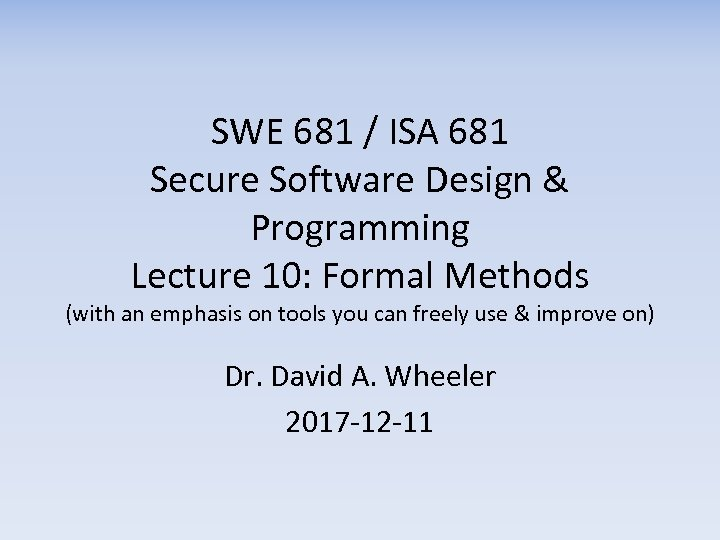SWE 681 / ISA 681 Secure Software Design & Programming Lecture 10: Formal Methods (with an emphasis on tools you can freely use & improve on) Dr. David A. Wheeler 2017 -12 -11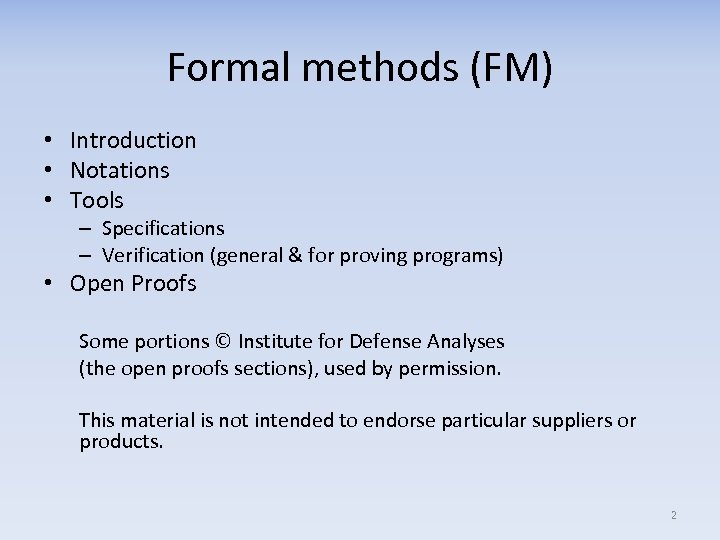Formal methods (FM) • Introduction • Notations • Tools – Specifications – Verification (general & for proving programs) • Open Proofs Some portions © Institute for Defense Analyses (the open proofs sections), used by permission. This material is not intended to endorse particular suppliers or products. 2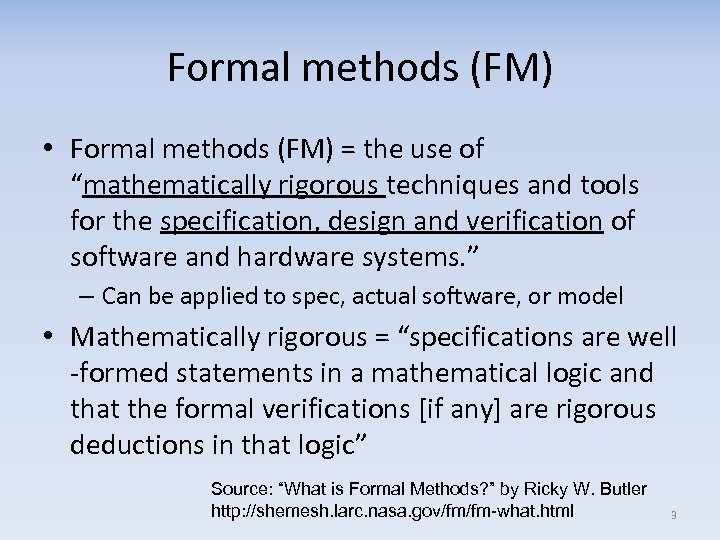Formal methods (FM) • Formal methods (FM) = the use of “mathematically rigorous techniques and tools for the specification, design and verification of software and hardware systems. ” – Can be applied to spec, actual software, or model • Mathematically rigorous = “specifications are well -formed statements in a mathematical logic and that the formal verifications [if any] are rigorous deductions in that logic” Source: “What is Formal Methods? ” by Ricky W. Butler http: //shemesh. larc. nasa. gov/fm/fm-what. html 3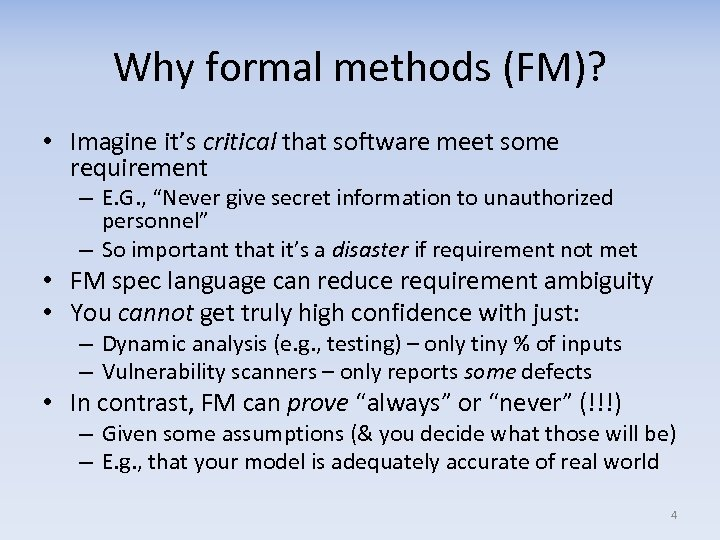Why formal methods (FM)? • Imagine it’s critical that software meet some requirement – E. G. , “Never give secret information to unauthorized personnel” – So important that it’s a disaster if requirement not met • FM spec language can reduce requirement ambiguity • You cannot get truly high confidence with just: – Dynamic analysis (e. g. , testing) – only tiny % of inputs – Vulnerability scanners – only reports some defects • In contrast, FM can prove “always” or “never” (!!!) – Given some assumptions (& you decide what those will be) – E. g. , that your model is adequately accurate of real world 4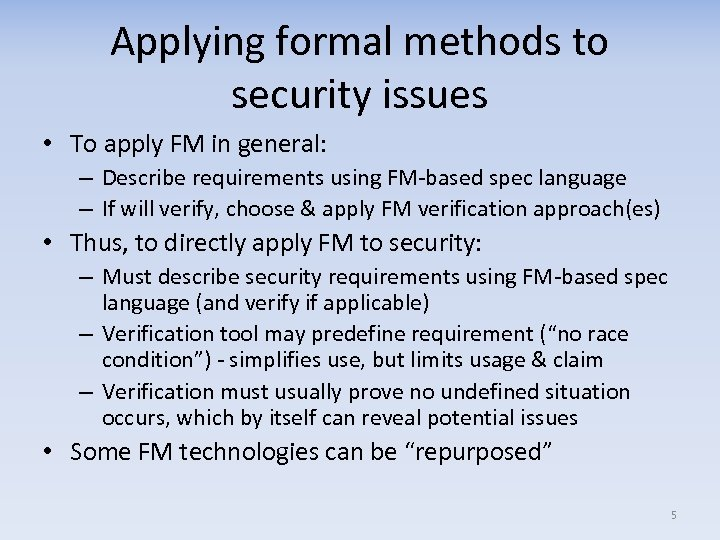Applying formal methods to security issues • To apply FM in general: – Describe requirements using FM-based spec language – If will verify, choose & apply FM verification approach(es) • Thus, to directly apply FM to security: – Must describe security requirements using FM-based spec language (and verify if applicable) – Verification tool may predefine requirement (“no race condition”) - simplifies use, but limits usage & claim – Verification must usually prove no undefined situation occurs, which by itself can reveal potential issues • Some FM technologies can be “repurposed” 5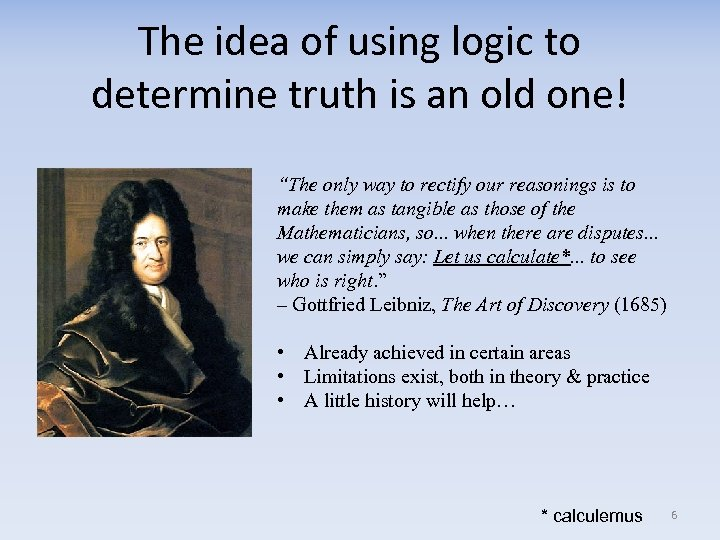The idea of using logic to determine truth is an old one! “The only way to rectify our reasonings is to make them as tangible as those of the Mathematicians, so. . . when there are disputes. . . we can simply say: Let us calculate*. . . to see who is right. ” – Gottfried Leibniz, The Art of Discovery (1685) • Already achieved in certain areas • Limitations exist, both in theory & practice • A little history will help… * calculemus 6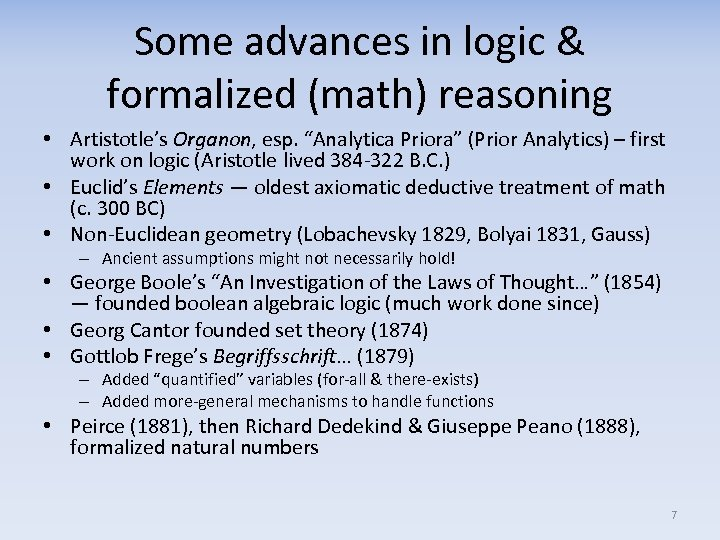Some advances in logic & formalized (math) reasoning • Artistotle’s Organon, esp. “Analytica Priora” (Prior Analytics) – first work on logic (Aristotle lived 384 -322 B. C. ) • Euclid’s Elements — oldest axiomatic deductive treatment of math (c. 300 BC) • Non-Euclidean geometry (Lobachevsky 1829, Bolyai 1831, Gauss) – Ancient assumptions might not necessarily hold! • George Boole’s “An Investigation of the Laws of Thought…” (1854) — founded boolean algebraic logic (much work done since) • Georg Cantor founded set theory (1874) • Gottlob Frege’s Begriffsschrift… (1879) – Added “quantified” variables (for-all & there-exists) – Added more-general mechanisms to handle functions • Peirce (1881), then Richard Dedekind & Giuseppe Peano (1888), formalized natural numbers 7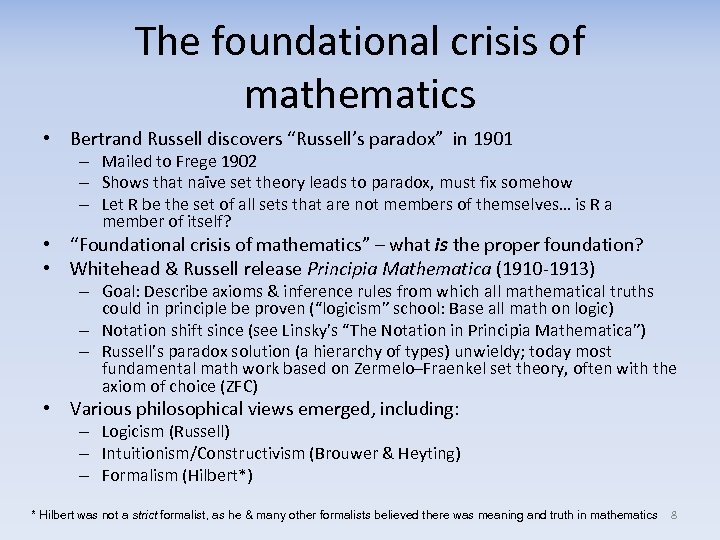The foundational crisis of mathematics • Bertrand Russell discovers “Russell’s paradox” in 1901 – Mailed to Frege 1902 – Shows that naïve set theory leads to paradox, must fix somehow – Let R be the set of all sets that are not members of themselves… is R a member of itself? • “Foundational crisis of mathematics” – what is the proper foundation? • Whitehead & Russell release Principia Mathematica (1910 -1913) – Goal: Describe axioms & inference rules from which all mathematical truths could in principle be proven (“logicism” school: Base all math on logic) – Notation shift since (see Linsky’s “The Notation in Principia Mathematica”) – Russell’s paradox solution (a hierarchy of types) unwieldy; today most fundamental math work based on Zermelo–Fraenkel set theory, often with the axiom of choice (ZFC) • Various philosophical views emerged, including: – Logicism (Russell) – Intuitionism/Constructivism (Brouwer & Heyting) – Formalism (Hilbert*) * Hilbert was not a strict formalist, as he & many other formalists believed there was meaning and truth in mathematics 8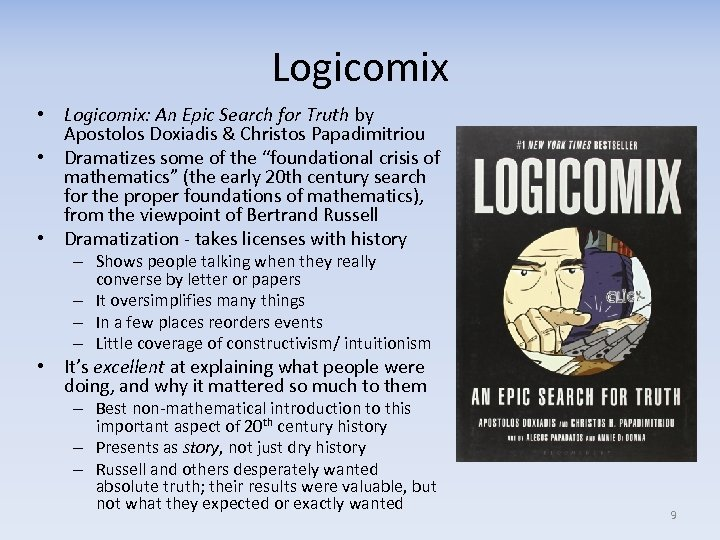Logicomix • Logicomix: An Epic Search for Truth by Apostolos Doxiadis & Christos Papadimitriou • Dramatizes some of the “foundational crisis of mathematics” (the early 20 th century search for the proper foundations of mathematics), from the viewpoint of Bertrand Russell • Dramatization - takes licenses with history – Shows people talking when they really converse by letter or papers – It oversimplifies many things – In a few places reorders events – Little coverage of constructivism/ intuitionism • It’s excellent at explaining what people were doing, and why it mattered so much to them – Best non-mathematical introduction to this important aspect of 20 th century history – Presents as story, not just dry history – Russell and others desperately wanted absolute truth; their results were valuable, but not what they expected or exactly wanted 9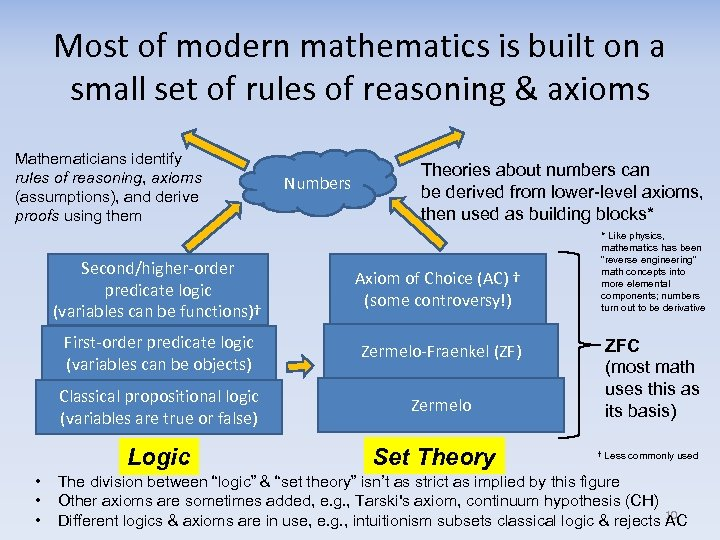Most of modern mathematics is built on a small set of rules of reasoning & axioms Mathematicians identify rules of reasoning, axioms (assumptions), and derive proofs using them Numbers Theories about numbers can be derived from lower-level axioms, then used as building blocks* Second/higher-order predicate logic (variables can be functions)† Axiom of Choice (AC) † (some controversy!) First-order predicate logic (variables can be objects) Zermelo-Fraenkel (ZF) Classical propositional logic (variables are true or false) Zermelo Logic • • • * Like physics, mathematics has been “reverse engineering” math concepts into more elemental components; numbers turn out to be derivative Set Theory ZFC (most math uses this as its basis) † Less commonly used The division between “logic” & “set theory” isn’t as strict as implied by this figure Other axioms are sometimes added, e. g. , Tarski's axiom, continuum hypothesis (CH) Different logics & axioms are in use, e. g. , intuitionism subsets classical logic & rejects 10 AC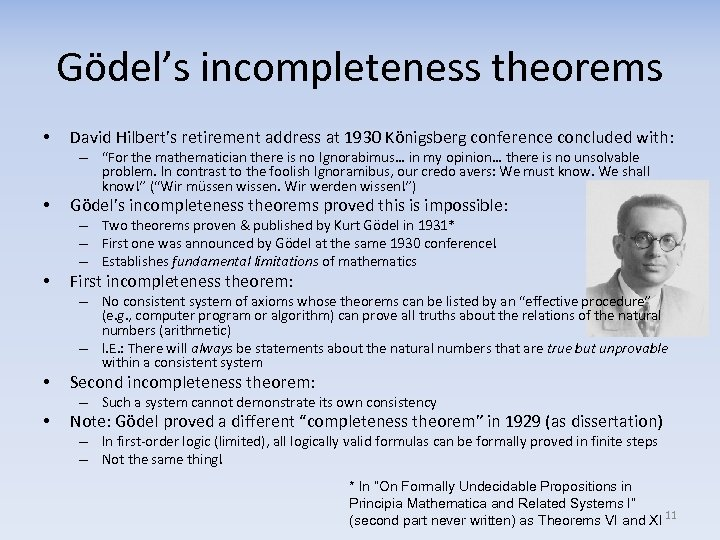Gödel’s incompleteness theorems • David Hilbert’s retirement address at 1930 Königsberg conference concluded with: – “For the mathematician there is no Ignorabimus… in my opinion… there is no unsolvable problem. In contrast to the foolish Ignoramibus, our credo avers: We must know. We shall know!” (“Wir müssen wissen. Wir werden wissen!”) • Gödel’s incompleteness theorems proved this is impossible: – Two theorems proven & published by Kurt Gödel in 1931* – First one was announced by Gödel at the same 1930 conference! – Establishes fundamental limitations of mathematics • First incompleteness theorem: – No consistent system of axioms whose theorems can be listed by an “effective procedure” (e. g. , computer program or algorithm) can prove all truths about the relations of the natural numbers (arithmetic) – I. E. : There will always be statements about the natural numbers that are true but unprovable within a consistent system • Second incompleteness theorem: – Such a system cannot demonstrate its own consistency • Note: Gödel proved a different “completeness theorem” in 1929 (as dissertation) – In first-order logic (limited), all logically valid formulas can be formally proved in finite steps – Not the same thing! * In “On Formally Undecidable Propositions in Principia Mathematica and Related Systems I” (second part never written) as Theorems VI and XI 11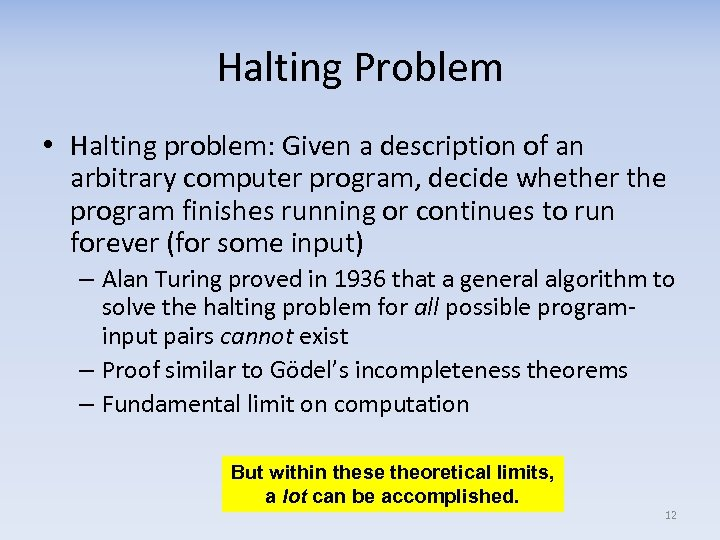Halting Problem • Halting problem: Given a description of an arbitrary computer program, decide whether the program finishes running or continues to run forever (for some input) – Alan Turing proved in 1936 that a general algorithm to solve the halting problem for all possible programinput pairs cannot exist – Proof similar to Gödel’s incompleteness theorems – Fundamental limit on computation But within these theoretical limits, a lot can be accomplished. 12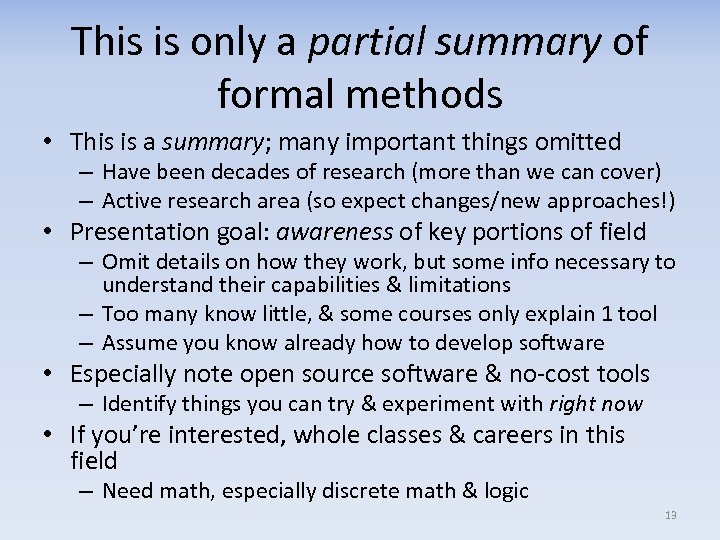This is only a partial summary of formal methods • This is a summary; many important things omitted – Have been decades of research (more than we can cover) – Active research area (so expect changes/new approaches!) • Presentation goal: awareness of key portions of field – Omit details on how they work, but some info necessary to understand their capabilities & limitations – Too many know little, & some courses only explain 1 tool – Assume you know already how to develop software • Especially note open source software & no-cost tools – Identify things you can try & experiment with right now • If you’re interested, whole classes & careers in this field – Need math, especially discrete math & logic 13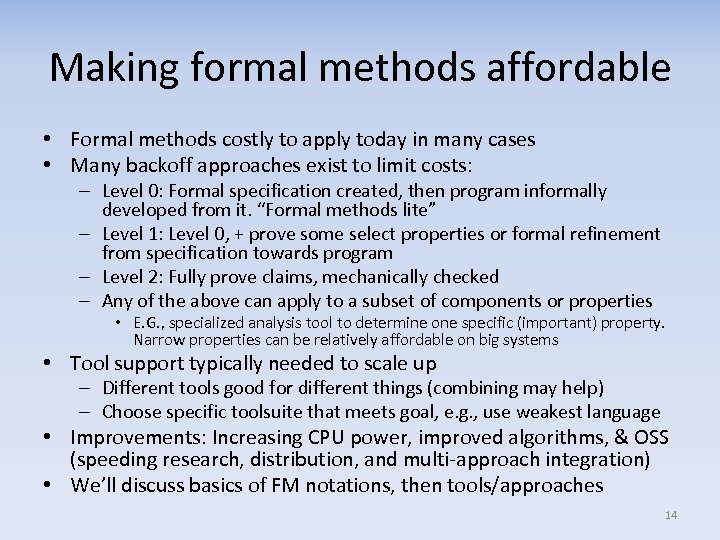Making formal methods affordable • Formal methods costly to apply today in many cases • Many backoff approaches exist to limit costs: – Level 0: Formal specification created, then program informally developed from it. “Formal methods lite” – Level 1: Level 0, + prove some select properties or formal refinement from specification towards program – Level 2: Fully prove claims, mechanically checked – Any of the above can apply to a subset of components or properties • E. G. , specialized analysis tool to determine one specific (important) property. Narrow properties can be relatively affordable on big systems • Tool support typically needed to scale up – Different tools good for different things (combining may help) – Choose specific toolsuite that meets goal, e. g. , use weakest language • Improvements: Increasing CPU power, improved algorithms, & OSS (speeding research, distribution, and multi-approach integration) • We’ll discuss basics of FM notations, then tools/approaches 14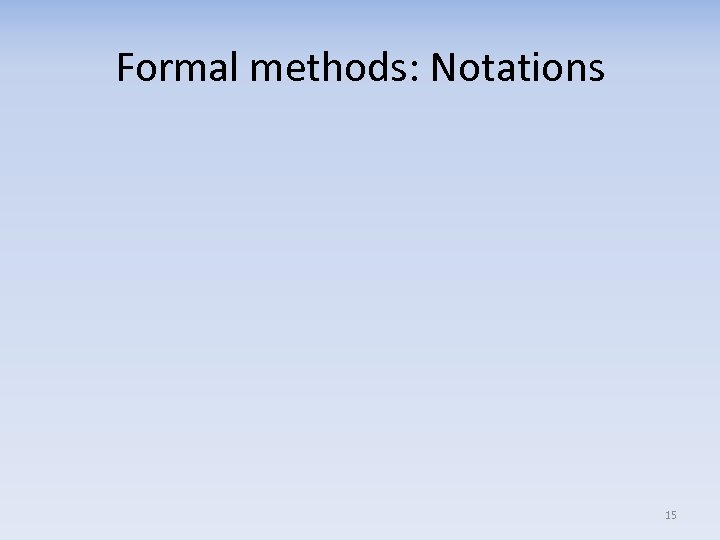Formal methods: Notations 15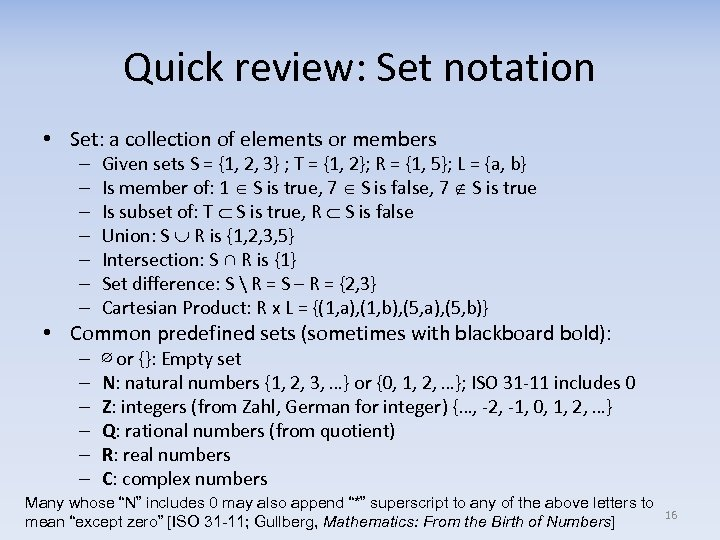Quick review: Set notation • Set: a collection of elements or members – – – – Given sets S = {1, 2, 3} ; T = {1, 2}; R = {1, 5}; L = {a, b} Is member of: 1 S is true, 7 S is false, 7 S is true Is subset of: T S is true, R S is false Union: S R is {1, 2, 3, 5} Intersection: S ∩ R is {1} Set difference: S R = S – R = {2, 3} Cartesian Product: R x L = {(1, a), (1, b), (5, a), (5, b)} • Common predefined sets (sometimes with blackboard bold): – – – ∅ or {}: Empty set N: natural numbers {1, 2, 3, …} or {0, 1, 2, …}; ISO 31 -11 includes 0 Z: integers (from Zahl, German for integer) {…, -2, -1, 0, 1, 2, …} Q: rational numbers (from quotient) R: real numbers C: complex numbers Many whose “N” includes 0 may also append “*” superscript to any of the above letters to mean “except zero” [ISO 31 -11; Gullberg, Mathematics: From the Birth of Numbers] 16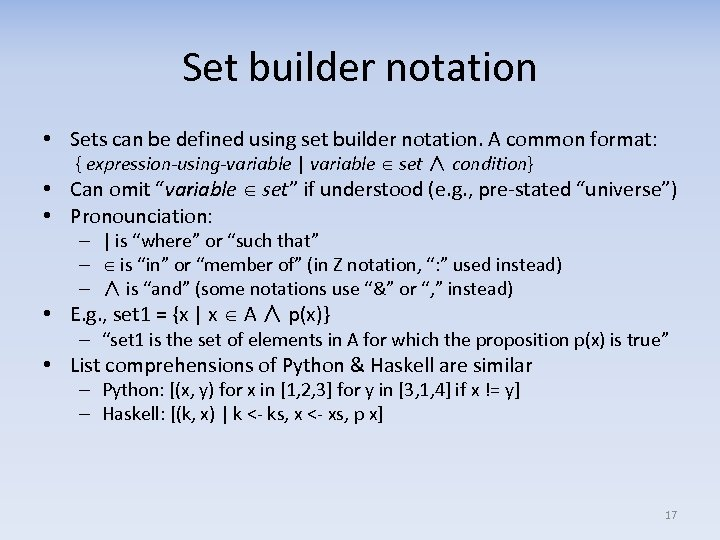Set builder notation • Sets can be defined using set builder notation. A common format: { expression-using-variable | variable set ∧ condition} • Can omit “variable set” if understood (e. g. , pre-stated “universe”) • Pronounciation: – | is “where” or “such that” – is “in” or “member of” (in Z notation, “: ” used instead) – ∧ is “and” (some notations use “&” or “, ” instead) • E. g. , set 1 = {x | x A ∧ p(x)} – “set 1 is the set of elements in A for which the proposition p(x) is true” • List comprehensions of Python & Haskell are similar – Python: [(x, y) for x in [1, 2, 3] for y in [3, 1, 4] if x != y] – Haskell: [(k, x) | k <- ks, x <- xs, p x] 17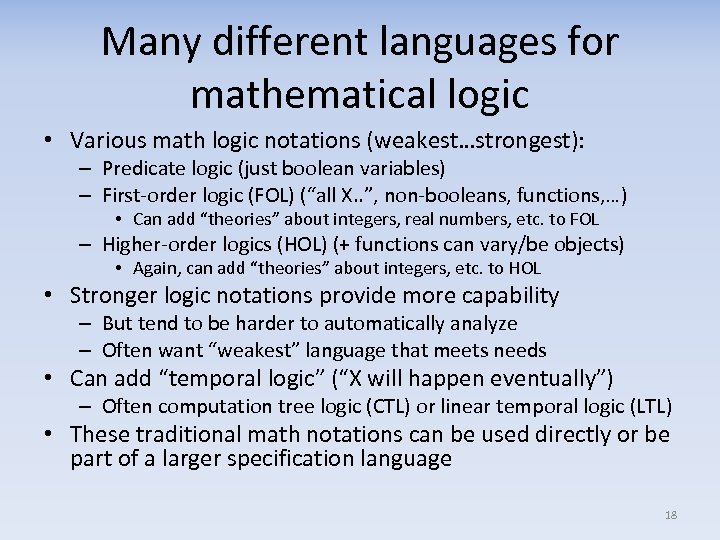Many different languages for mathematical logic • Various math logic notations (weakest…strongest): – Predicate logic (just boolean variables) – First-order logic (FOL) (“all X. . ”, non-booleans, functions, …) • Can add “theories” about integers, real numbers, etc. to FOL – Higher-order logics (HOL) (+ functions can vary/be objects) • Again, can add “theories” about integers, etc. to HOL • Stronger logic notations provide more capability – But tend to be harder to automatically analyze – Often want “weakest” language that meets needs • Can add “temporal logic” (“X will happen eventually”) – Often computation tree logic (CTL) or linear temporal logic (LTL) • These traditional math notations can be used directly or be part of a larger specification language 18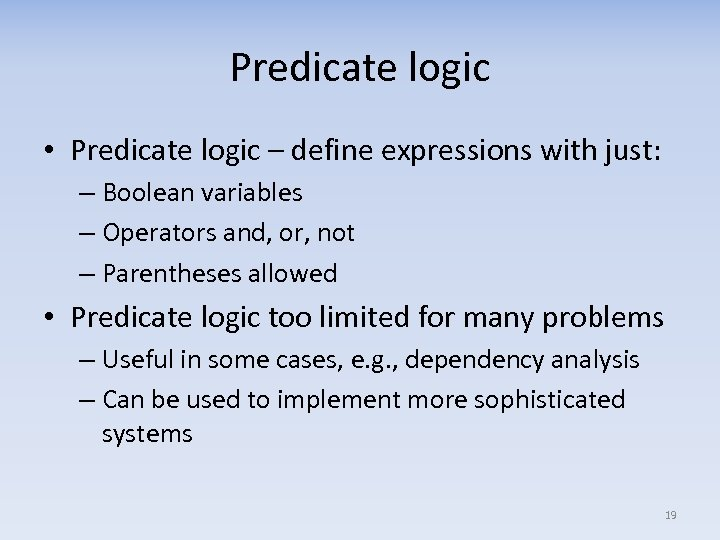Predicate logic • Predicate logic – define expressions with just: – Boolean variables – Operators and, or, not – Parentheses allowed • Predicate logic too limited for many problems – Useful in some cases, e. g. , dependency analysis – Can be used to implement more sophisticated systems 19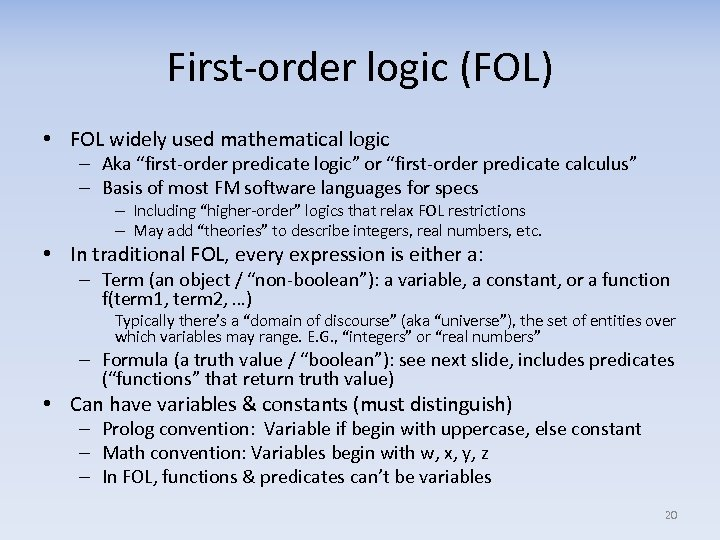First-order logic (FOL) • FOL widely used mathematical logic – Aka “first-order predicate logic” or “first-order predicate calculus” – Basis of most FM software languages for specs – Including “higher-order” logics that relax FOL restrictions – May add “theories” to describe integers, real numbers, etc. • In traditional FOL, every expression is either a: – Term (an object / “non-boolean”): a variable, a constant, or a function f(term 1, term 2, …) Typically there’s a “domain of discourse” (aka “universe”), the set of entities over which variables may range. E. G. , “integers” or “real numbers” – Formula (a truth value / “boolean”): see next slide, includes predicates (“functions” that return truth value) • Can have variables & constants (must distinguish) – Prolog convention: Variable if begin with uppercase, else constant – Math convention: Variables begin with w, x, y, z – In FOL, functions & predicates can’t be variables 20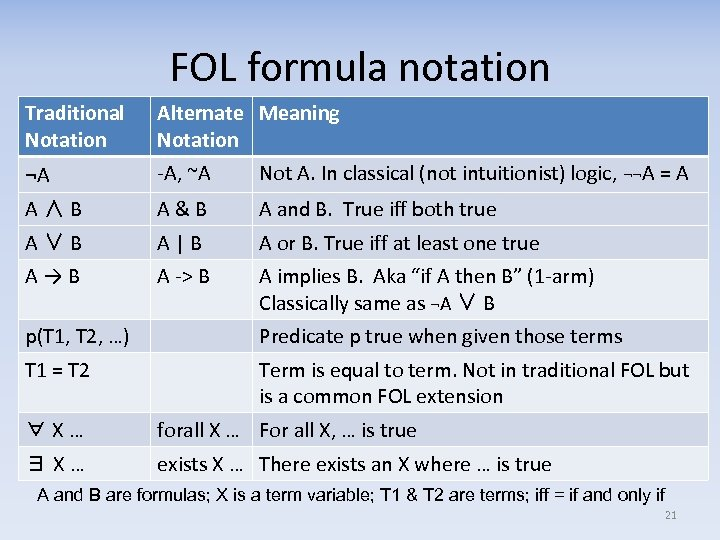FOL formula notation Traditional Notation Alternate Meaning Notation ¬A -A, ~A Not A. In classical (not intuitionist) logic, ¬¬A = A A ∧ B A & B A and B. True iff both true A ∨ B A | B A or B. True iff at least one true A → B A -> B A implies B. Aka “if A then B” (1 -arm) Classically same as ¬A ∨ B p(T 1, T 2, …) Predicate p true when given those terms T 1 = T 2 Term is equal to term. Not in traditional FOL but is a common FOL extension ∀ X … forall X … For all X, … is true ∃ X … exists X … There exists an X where … is true A and B are formulas; X is a term variable; T 1 & T 2 are terms; iff = if and only if 21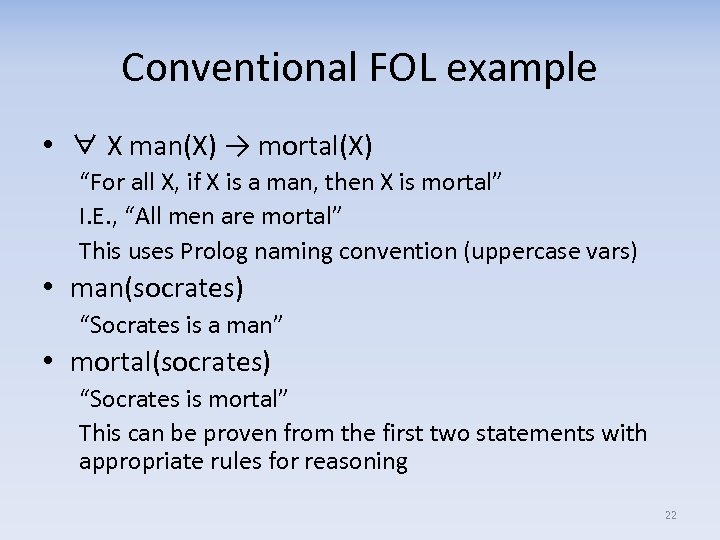Conventional FOL example • ∀ X man(X) → mortal(X) “For all X, if X is a man, then X is mortal” I. E. , “All men are mortal” This uses Prolog naming convention (uppercase vars) • man(socrates) “Socrates is a man” • mortal(socrates) “Socrates is mortal” This can be proven from the first two statements with appropriate rules for reasoning 22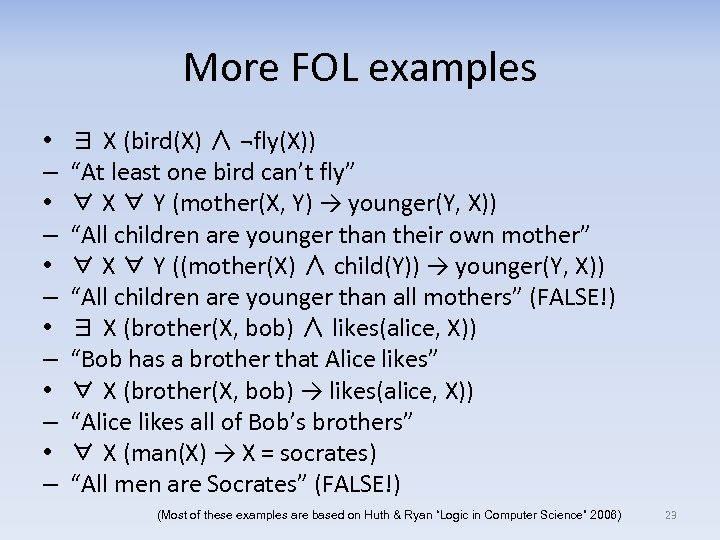More FOL examples • – • – • – ∃ X (bird(X) ∧ ¬fly(X)) “At least one bird can’t fly” ∀ X ∀ Y (mother(X, Y) → younger(Y, X)) “All children are younger than their own mother” ∀ X ∀ Y ((mother(X) ∧ child(Y)) → younger(Y, X)) “All children are younger than all mothers” (FALSE!) ∃ X (brother(X, bob) ∧ likes(alice, X)) “Bob has a brother that Alice likes” ∀ X (brother(X, bob) → likes(alice, X)) “Alice likes all of Bob’s brothers” ∀ X (man(X) → X = socrates) “All men are Socrates” (FALSE!) (Most of these examples are based on Huth & Ryan “Logic in Computer Science” 2006) 23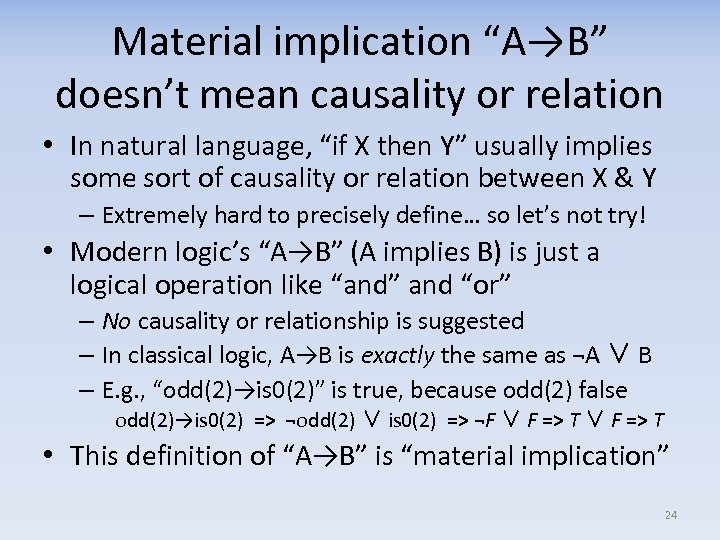Material implication “A→B” doesn’t mean causality or relation • In natural language, “if X then Y” usually implies some sort of causality or relation between X & Y – Extremely hard to precisely define… so let’s not try! • Modern logic’s “A→B” (A implies B) is just a logical operation like “and” and “or” – No causality or relationship is suggested – In classical logic, A→B is exactly the same as ¬A ∨ B – E. g. , “odd(2)→is 0(2)” is true, because odd(2) false odd(2)→is 0(2) => ¬odd(2) ∨ is 0(2) => ¬F ∨ F => T • This definition of “A→B” is “material implication” 24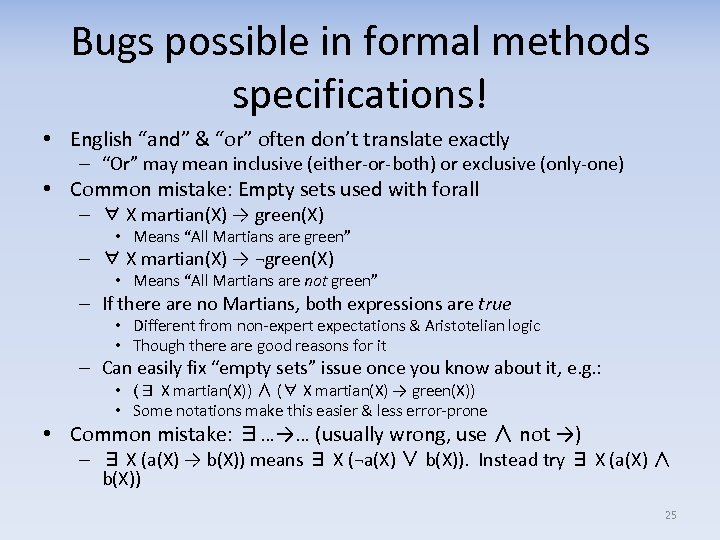Bugs possible in formal methods specifications! • English “and” & “or” often don’t translate exactly – “Or” may mean inclusive (either-or-both) or exclusive (only-one) • Common mistake: Empty sets used with forall – ∀ X martian(X) → green(X) • Means “All Martians are green” – ∀ X martian(X) → ¬green(X) • Means “All Martians are not green” – If there are no Martians, both expressions are true • Different from non-expert expectations & Aristotelian logic • Though there are good reasons for it – Can easily fix “empty sets” issue once you know about it, e. g. : • (∃ X martian(X)) ∧ (∀ X martian(X) → green(X)) • Some notations make this easier & less error-prone • Common mistake: ∃…→… (usually wrong, use ∧ not →) – ∃ X (a(X) → b(X)) means ∃ X (¬a(X) ∨ b(X)). Instead try ∃ X (a(X) ∧ b(X)) 25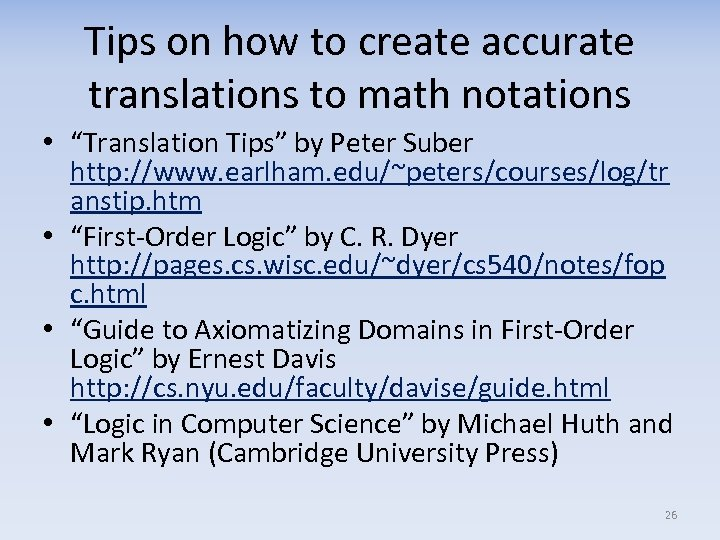Tips on how to create accurate translations to math notations • “Translation Tips” by Peter Suber http: //www. earlham. edu/~peters/courses/log/tr anstip. htm • “First-Order Logic” by C. R. Dyer http: //pages. cs. wisc. edu/~dyer/cs 540/notes/fop c. html • “Guide to Axiomatizing Domains in First-Order Logic” by Ernest Davis http: //cs. nyu. edu/faculty/davise/guide. html • “Logic in Computer Science” by Michael Huth and Mark Ryan (Cambridge University Press) 26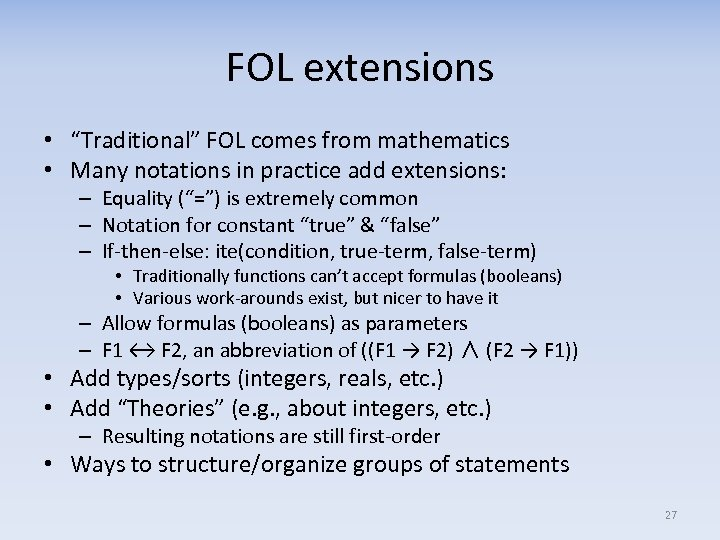FOL extensions • “Traditional” FOL comes from mathematics • Many notations in practice add extensions: – Equality (“=”) is extremely common – Notation for constant “true” & “false” – If-then-else: ite(condition, true-term, false-term) • Traditionally functions can’t accept formulas (booleans) • Various work-arounds exist, but nicer to have it – Allow formulas (booleans) as parameters – F 1 ↔ F 2, an abbreviation of ((F 1 → F 2) ∧ (F 2 → F 1)) • Add types/sorts (integers, reals, etc. ) • Add “Theories” (e. g. , about integers, etc. ) – Resulting notations are still first-order • Ways to structure/organize groups of statements 27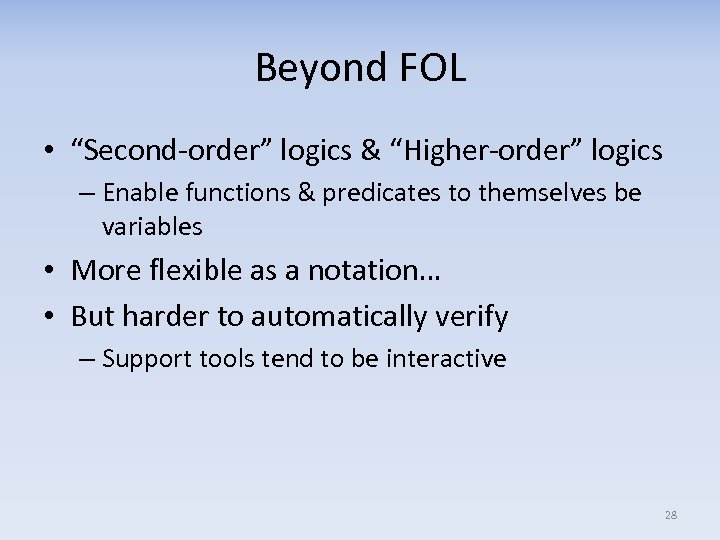Beyond FOL • “Second-order” logics & “Higher-order” logics – Enable functions & predicates to themselves be variables • More flexible as a notation… • But harder to automatically verify – Support tools tend to be interactive 28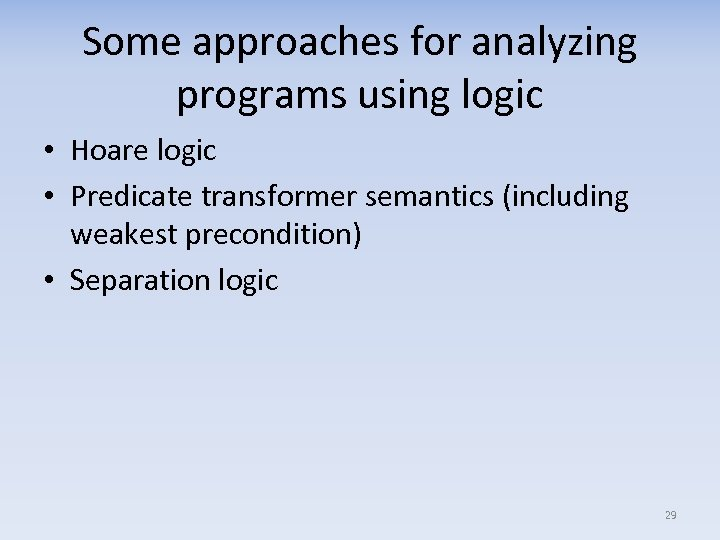Some approaches for analyzing programs using logic • Hoare logic • Predicate transformer semantics (including weakest precondition) • Separation logic 29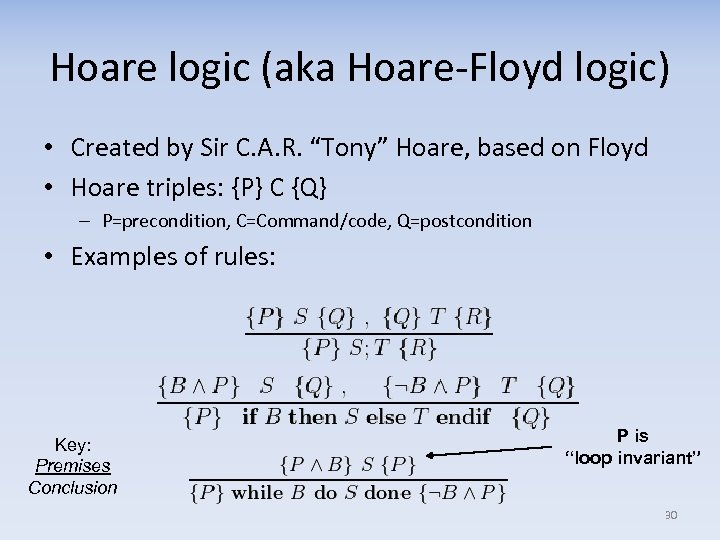Hoare logic (aka Hoare-Floyd logic) • Created by Sir C. A. R. “Tony” Hoare, based on Floyd • Hoare triples: {P} C {Q} – P=precondition, C=Command/code, Q=postcondition • Examples of rules: Key: Premises Conclusion P is “loop invariant” 30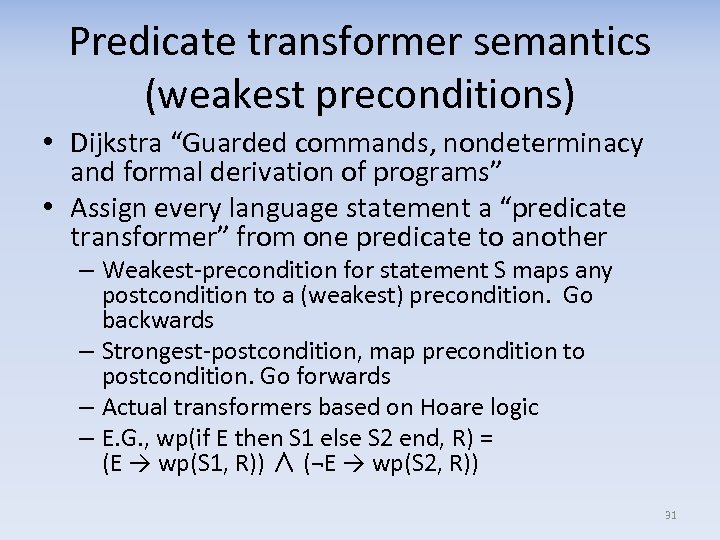Predicate transformer semantics (weakest preconditions) • Dijkstra “Guarded commands, nondeterminacy and formal derivation of programs” • Assign every language statement a “predicate transformer” from one predicate to another – Weakest-precondition for statement S maps any postcondition to a (weakest) precondition. Go backwards – Strongest-postcondition, map precondition to postcondition. Go forwards – Actual transformers based on Hoare logic – E. G. , wp(if E then S 1 else S 2 end, R) = (E → wp(S 1, R)) ∧ (¬E → wp(S 2, R)) 31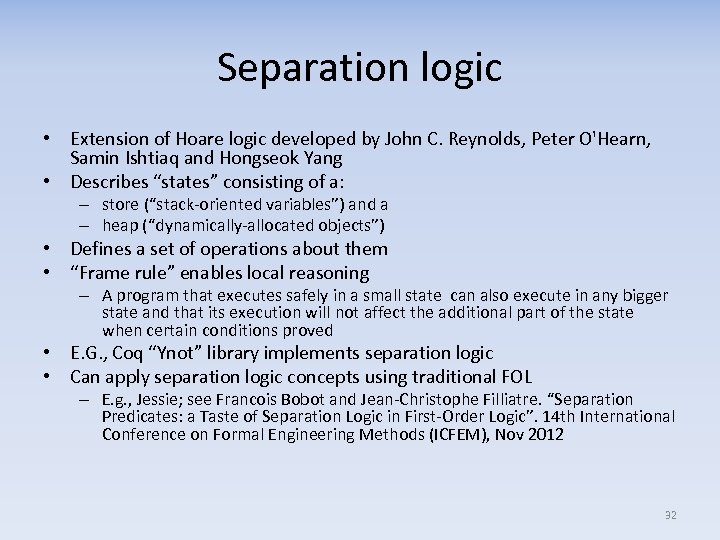Separation logic • Extension of Hoare logic developed by John C. Reynolds, Peter O'Hearn, Samin Ishtiaq and Hongseok Yang • Describes “states” consisting of a: – store (“stack-oriented variables”) and a – heap (“dynamically-allocated objects”) • Defines a set of operations about them • “Frame rule” enables local reasoning – A program that executes safely in a small state can also execute in any bigger state and that its execution will not affect the additional part of the state when certain conditions proved • E. G. , Coq “Ynot” library implements separation logic • Can apply separation logic concepts using traditional FOL – E. g. , Jessie; see Francois Bobot and Jean-Christophe Filliatre. “Separation Predicates: a Taste of Separation Logic in First-Order Logic”. 14 th International Conference on Formal Engineering Methods (ICFEM), Nov 2012 32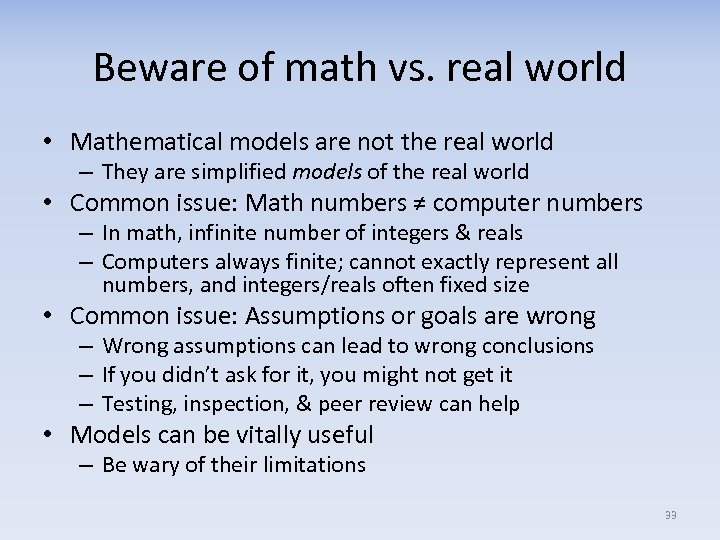Beware of math vs. real world • Mathematical models are not the real world – They are simplified models of the real world • Common issue: Math numbers ≠ computer numbers – In math, infinite number of integers & reals – Computers always finite; cannot exactly represent all numbers, and integers/reals often fixed size • Common issue: Assumptions or goals are wrong – Wrong assumptions can lead to wrong conclusions – If you didn’t ask for it, you might not get it – Testing, inspection, & peer review can help • Models can be vitally useful – Be wary of their limitations 33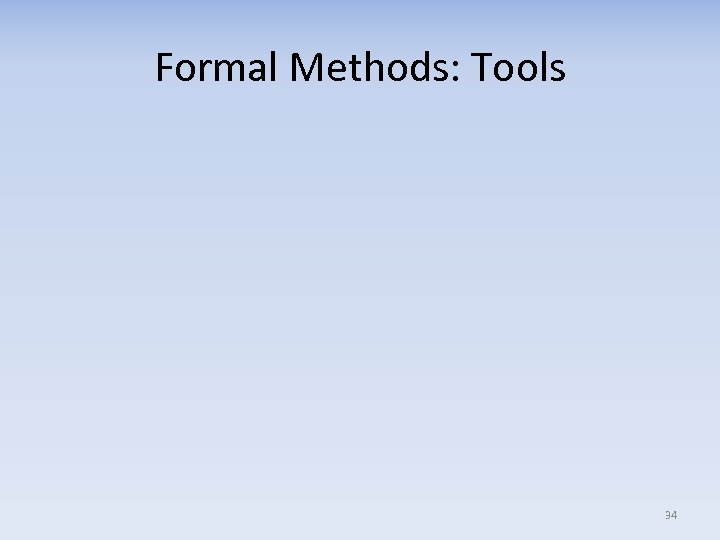Formal Methods: Tools 34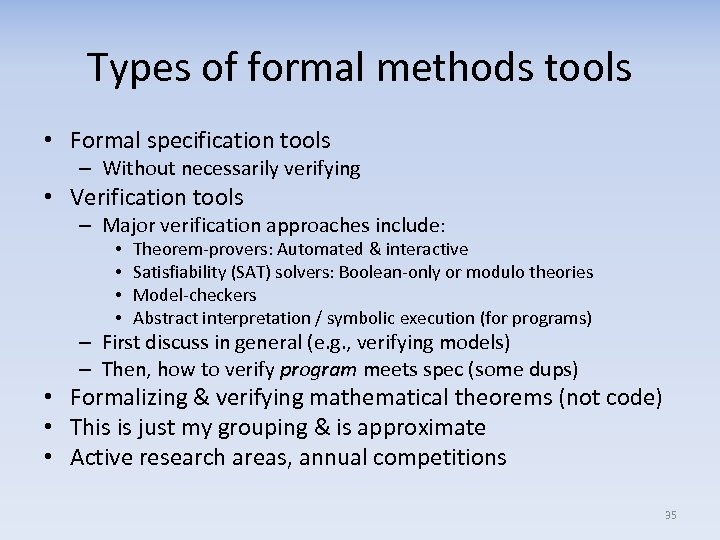Types of formal methods tools • Formal specification tools – Without necessarily verifying • Verification tools – Major verification approaches include: • • Theorem-provers: Automated & interactive Satisfiability (SAT) solvers: Boolean-only or modulo theories Model-checkers Abstract interpretation / symbolic execution (for programs) – First discuss in general (e. g. , verifying models) – Then, how to verify program meets spec (some dups) • Formalizing & verifying mathematical theorems (not code) • This is just my grouping & is approximate • Active research areas, annual competitions 35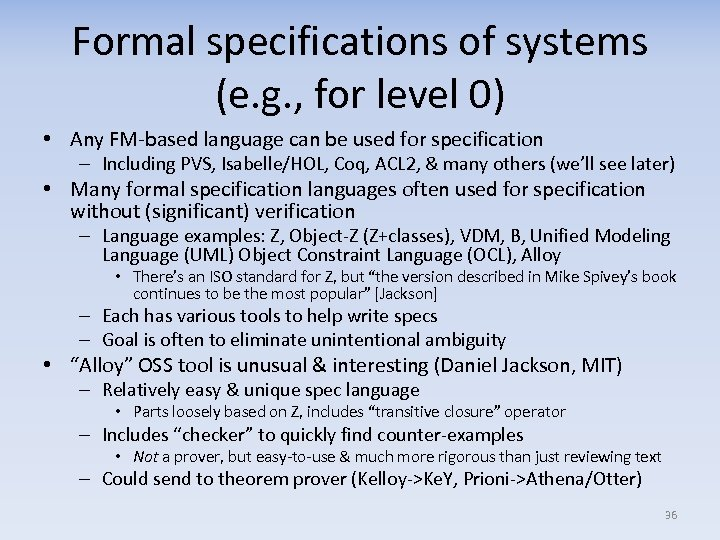Formal specifications of systems (e. g. , for level 0) • Any FM-based language can be used for specification – Including PVS, Isabelle/HOL, Coq, ACL 2, & many others (we’ll see later) • Many formal specification languages often used for specification without (significant) verification – Language examples: Z, Object-Z (Z+classes), VDM, B, Unified Modeling Language (UML) Object Constraint Language (OCL), Alloy • There’s an ISO standard for Z, but “the version described in Mike Spivey’s book continues to be the most popular” [Jackson] – Each has various tools to help write specs – Goal is often to eliminate unintentional ambiguity • “Alloy” OSS tool is unusual & interesting (Daniel Jackson, MIT) – Relatively easy & unique spec language • Parts loosely based on Z, includes “transitive closure” operator – Includes “checker” to quickly find counter-examples • Not a prover, but easy-to-use & much more rigorous than just reviewing text – Could send to theorem prover (Kelloy->Ke. Y, Prioni->Athena/Otter) 36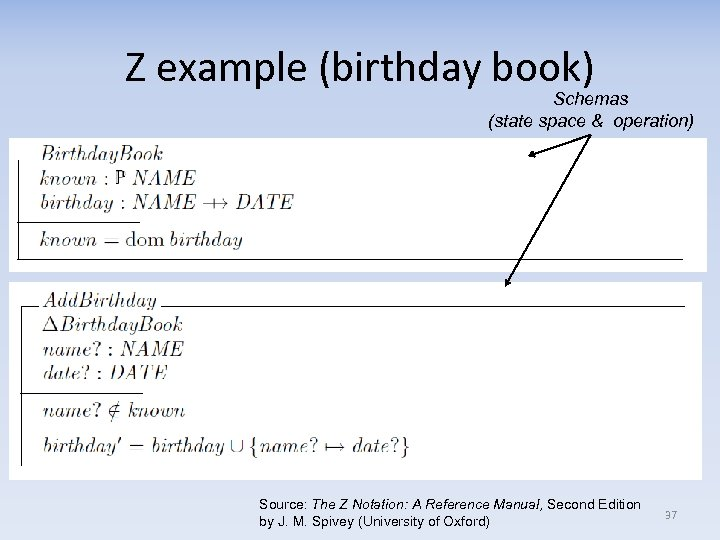Z example (birthday book) Schemas (state space & operation) Source: The Z Notation: A Reference Manual, Second Edition by J. M. Spivey (University of Oxford) 37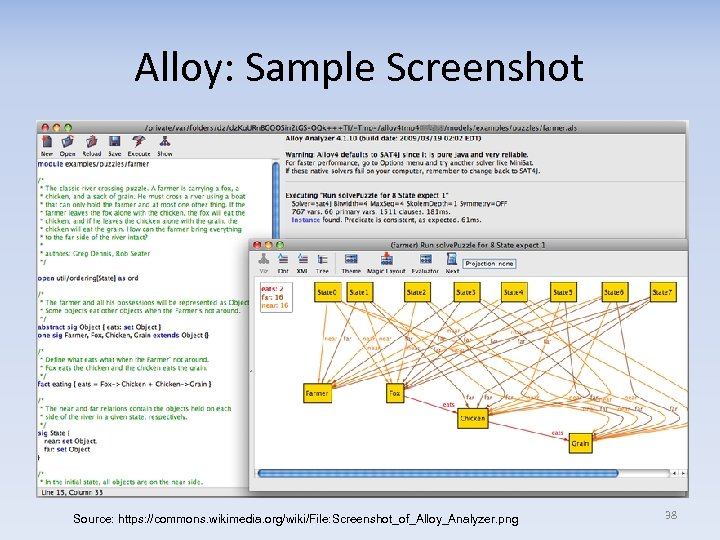Alloy: Sample Screenshot Source: https: //commons. wikimedia. org/wiki/File: Screenshot_of_Alloy_Analyzer. png 38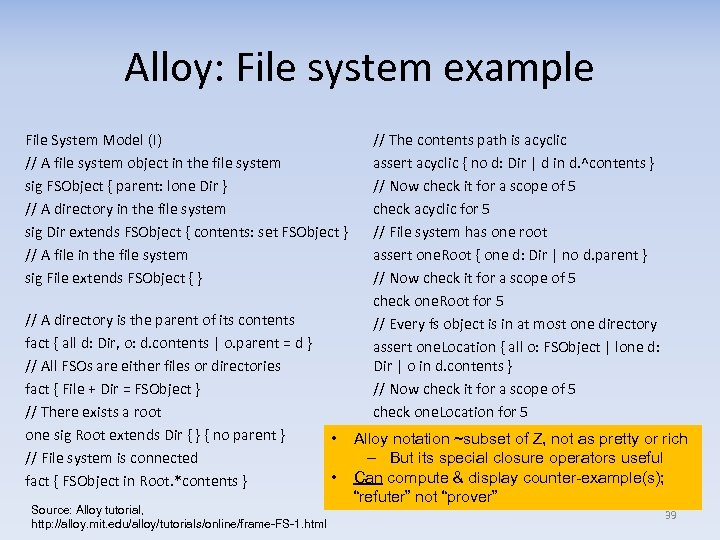Alloy: File system example File System Model (I) // A file system object in the file system sig FSObject { parent: lone Dir } // A directory in the file system sig Dir extends FSObject { contents: set FSObject } // A file in the file system sig File extends FSObject { } // A directory is the parent of its contents fact { all d: Dir, o: d. contents | o. parent = d } // All FSOs are either files or directories fact { File + Dir = FSObject } // There exists a root one sig Root extends Dir { } { no parent } // File system is connected fact { FSObject in Root. *contents } Source: Alloy tutorial, http: //alloy. mit. edu/alloy/tutorials/online/frame-FS-1. html • • // The contents path is acyclic assert acyclic { no d: Dir | d in d. ^contents } // Now check it for a scope of 5 check acyclic for 5 // File system has one root assert one. Root { one d: Dir | no d. parent } // Now check it for a scope of 5 check one. Root for 5 // Every fs object is in at most one directory assert one. Location { all o: FSObject | lone d: Dir | o in d. contents } // Now check it for a scope of 5 check one. Location for 5 Alloy notation ~subset of Z, not as pretty or rich – But its special closure operators useful Can compute & display counter-example(s); “refuter” not “prover” 39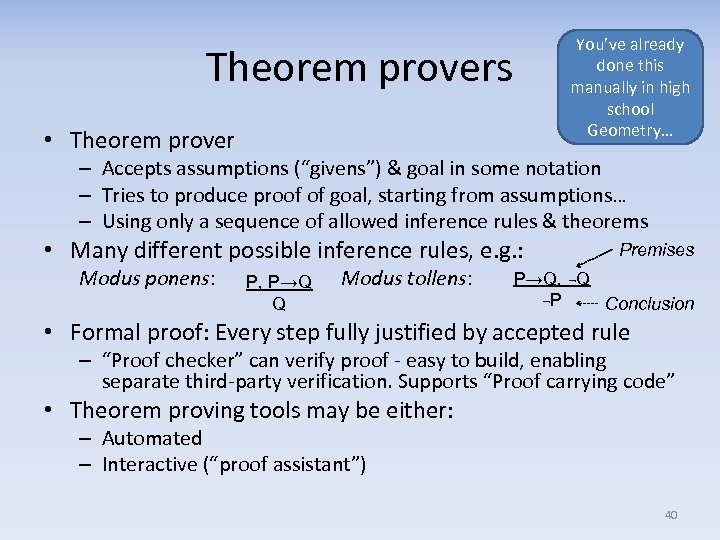Theorem provers • Theorem prover You’ve already done this manually in high school Geometry… – Accepts assumptions (“givens”) & goal in some notation – Tries to produce proof of goal, starting from assumptions… – Using only a sequence of allowed inference rules & theorems • Many different possible inference rules, e. g. : Modus ponens: P, P→Q Q Modus tollens: Premises P→Q, ¬Q ¬P Conclusion • Formal proof: Every step fully justified by accepted rule – “Proof checker” can verify proof - easy to build, enabling separate third-party verification. Supports “Proof carrying code” • Theorem proving tools may be either: – Automated – Interactive (“proof assistant”) 40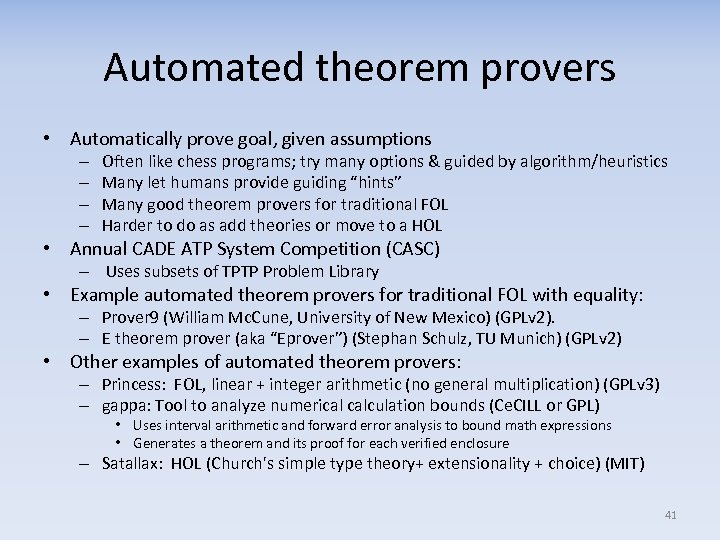Automated theorem provers • Automatically prove goal, given assumptions – – Often like chess programs; try many options & guided by algorithm/heuristics Many let humans provide guiding “hints” Many good theorem provers for traditional FOL Harder to do as add theories or move to a HOL • Annual CADE ATP System Competition (CASC) – Uses subsets of TPTP Problem Library • Example automated theorem provers for traditional FOL with equality: – Prover 9 (William Mc. Cune, University of New Mexico) (GPLv 2). – E theorem prover (aka “Eprover”) (Stephan Schulz, TU Munich) (GPLv 2) • Other examples of automated theorem provers: – Princess: FOL, linear + integer arithmetic (no general multiplication) (GPLv 3) – gappa: Tool to analyze numerical calculation bounds (Ce. CILL or GPL) • Uses interval arithmetic and forward error analysis to bound math expressions • Generates a theorem and its proof for each verified enclosure – Satallax: HOL (Church's simple type theory+ extensionality + choice) (MIT) 41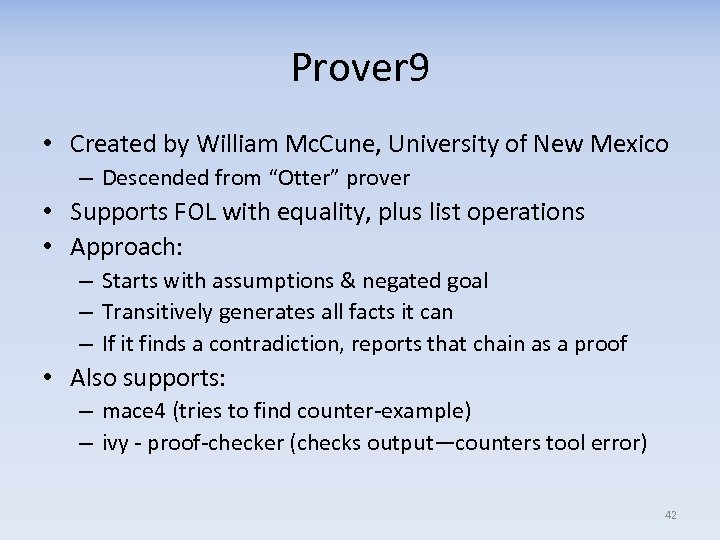Prover 9 • Created by William Mc. Cune, University of New Mexico – Descended from “Otter” prover • Supports FOL with equality, plus list operations • Approach: – Starts with assumptions & negated goal – Transitively generates all facts it can – If it finds a contradiction, reports that chain as a proof • Also supports: – mace 4 (tries to find counter-example) – ivy - proof-checker (checks output—counters tool error) 42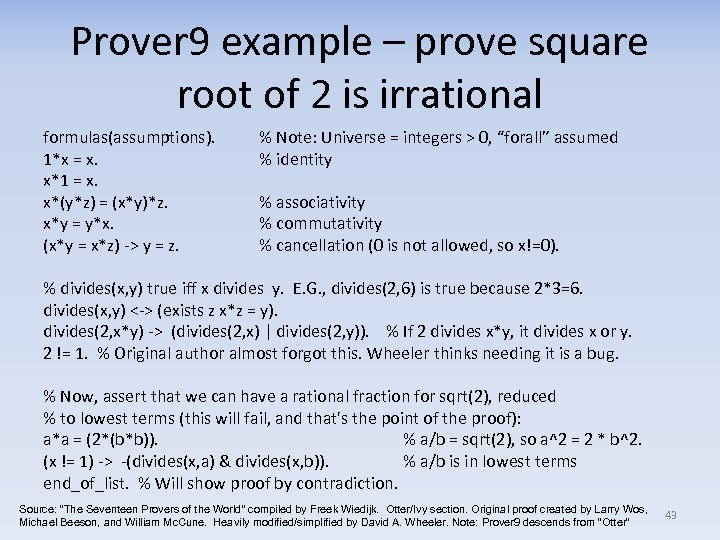Prover 9 example – prove square root of 2 is irrational formulas(assumptions). 1*x = x. x*1 = x. x*(y*z) = (x*y)*z. x*y = y*x. (x*y = x*z) -> y = z. % Note: Universe = integers > 0, “forall” assumed % identity % associativity % commutativity % cancellation (0 is not allowed, so x!=0). % divides(x, y) true iff x divides y. E. G. , divides(2, 6) is true because 2*3=6. divides(x, y) <-> (exists z x*z = y). divides(2, x*y) -> (divides(2, x) | divides(2, y)). % If 2 divides x*y, it divides x or y. 2 != 1. % Original author almost forgot this. Wheeler thinks needing it is a bug. % Now, assert that we can have a rational fraction for sqrt(2), reduced % to lowest terms (this will fail, and that's the point of the proof): a*a = (2*(b*b)). % a/b = sqrt(2), so a^2 = 2 * b^2. (x != 1) -> -(divides(x, a) & divides(x, b)). % a/b is in lowest terms end_of_list. % Will show proof by contradiction. Source: “The Seventeen Provers of the World” compiled by Freek Wiedijk. Otter/Ivy section. Original proof created by Larry Wos, Michael Beeson, and William Mc. Cune. Heavily modified/simplified by David A. Wheeler. Note: Prover 9 descends from “Otter” 43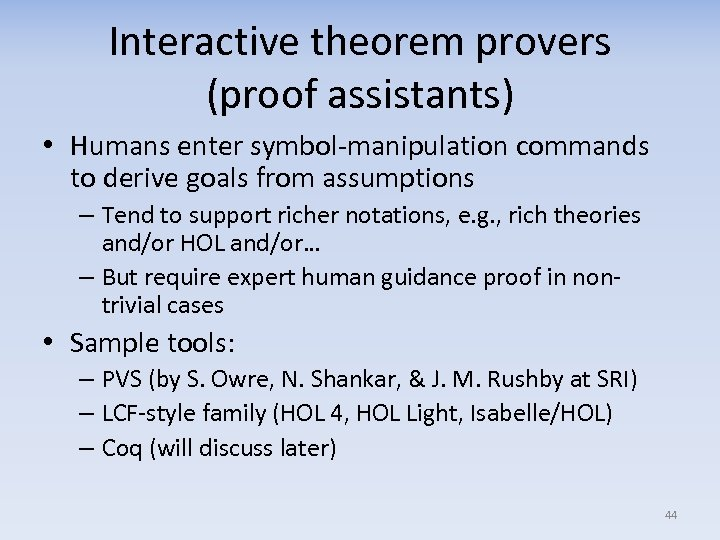Interactive theorem provers (proof assistants) • Humans enter symbol-manipulation commands to derive goals from assumptions – Tend to support richer notations, e. g. , rich theories and/or HOL and/or… – But require expert human guidance proof in nontrivial cases • Sample tools: – PVS (by S. Owre, N. Shankar, & J. M. Rushby at SRI) – LCF-style family (HOL 4, HOL Light, Isabelle/HOL) – Coq (will discuss later) 44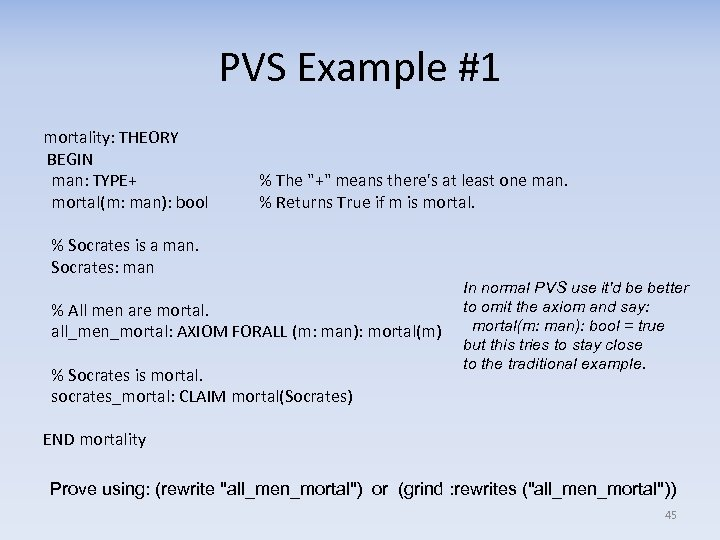PVS Example #1 mortality: THEORY BEGIN man: TYPE+ mortal(m: man): bool % The "+" means there's at least one man. % Returns True if m is mortal. % Socrates is a man. Socrates: man % All men are mortal. all_men_mortal: AXIOM FORALL (m: man): mortal(m) % Socrates is mortal. socrates_mortal: CLAIM mortal(Socrates) In normal PVS use it'd be better to omit the axiom and say: mortal(m: man): bool = true but this tries to stay close to the traditional example. END mortality Prove using: (rewrite "all_men_mortal") or (grind : rewrites ("all_men_mortal")) 45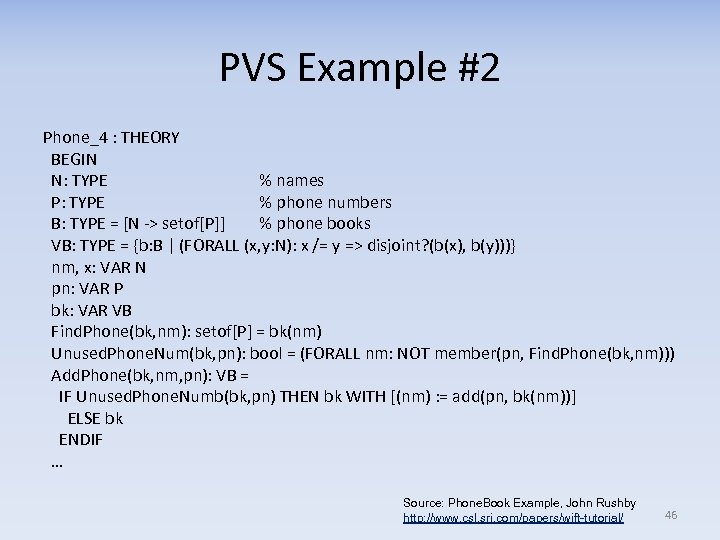PVS Example #2 Phone_4 : THEORY BEGIN N: TYPE % names P: TYPE % phone numbers B: TYPE = [N -> setof[P]] % phone books VB: TYPE = {b: B | (FORALL (x, y: N): x /= y => disjoint? (b(x), b(y)))} nm, x: VAR N pn: VAR P bk: VAR VB Find. Phone(bk, nm): setof[P] = bk(nm) Unused. Phone. Num(bk, pn): bool = (FORALL nm: NOT member(pn, Find. Phone(bk, nm))) Add. Phone(bk, nm, pn): VB = IF Unused. Phone. Numb(bk, pn) THEN bk WITH [(nm) : = add(pn, bk(nm))] ELSE bk ENDIF … Source: Phone. Book Example, John Rushby http: //www. csl. sri. com/papers/wift-tutorial/ 46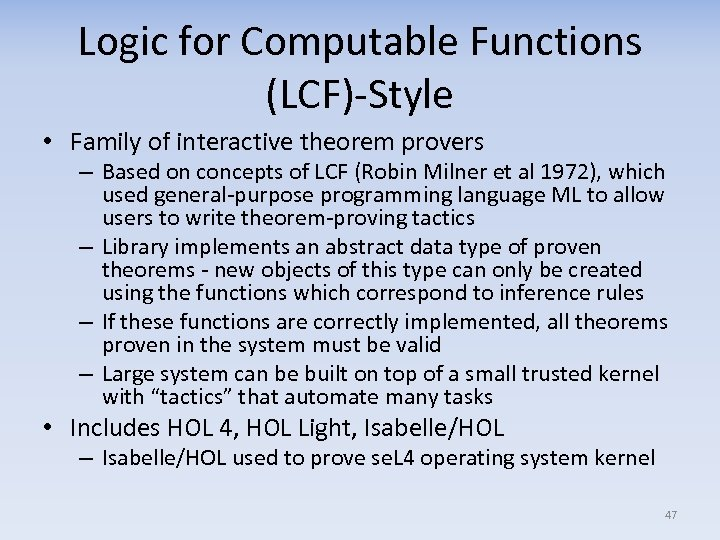Logic for Computable Functions (LCF)-Style • Family of interactive theorem provers – Based on concepts of LCF (Robin Milner et al 1972), which used general-purpose programming language ML to allow users to write theorem-proving tactics – Library implements an abstract data type of proven theorems - new objects of this type can only be created using the functions which correspond to inference rules – If these functions are correctly implemented, all theorems proven in the system must be valid – Large system can be built on top of a small trusted kernel with “tactics” that automate many tasks • Includes HOL 4, HOL Light, Isabelle/HOL – Isabelle/HOL used to prove se. L 4 operating system kernel 47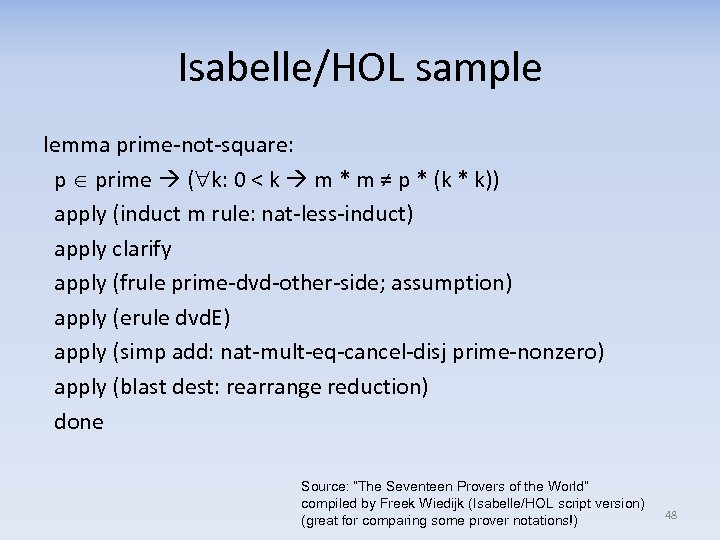Isabelle/HOL sample lemma prime-not-square: p prime ( k: 0 < k m * m ≠ p * (k * k)) apply (induct m rule: nat-less-induct) apply clarify apply (frule prime-dvd-other-side; assumption) apply (erule dvd. E) apply (simp add: nat-mult-eq-cancel-disj prime-nonzero) apply (blast dest: rearrange reduction) done Source: “The Seventeen Provers of the World” compiled by Freek Wiedijk (Isabelle/HOL script version) (great for comparing some prover notations!) 48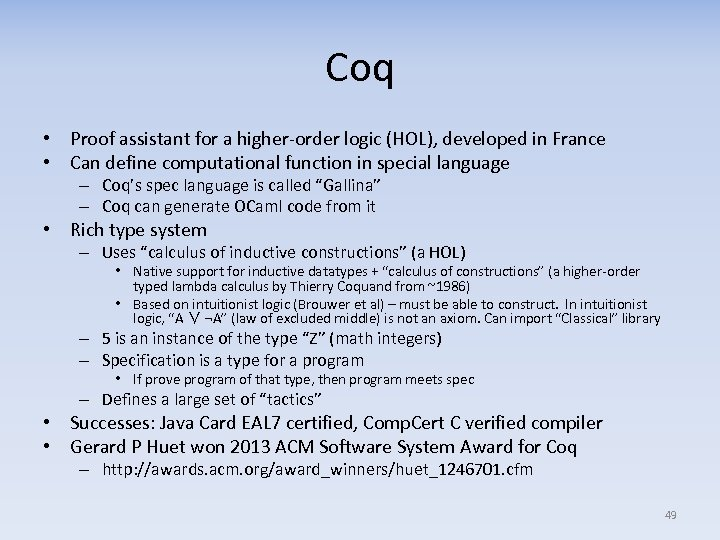Coq • Proof assistant for a higher-order logic (HOL), developed in France • Can define computational function in special language – Coq’s spec language is called “Gallina” – Coq can generate OCaml code from it • Rich type system – Uses “calculus of inductive constructions” (a HOL) • Native support for inductive datatypes + “calculus of constructions” (a higher-order typed lambda calculus by Thierry Coquand from ~1986) • Based on intuitionist logic (Brouwer et al) – must be able to construct. In intuitionist logic, “A ∨ ¬A” (law of excluded middle) is not an axiom. Can import “Classical” library – 5 is an instance of the type “Z” (math integers) – Specification is a type for a program • If prove program of that type, then program meets spec – Defines a large set of “tactics” • Successes: Java Card EAL 7 certified, Comp. Cert C verified compiler • Gerard P Huet won 2013 ACM Software System Award for Coq – http: //awards. acm. org/award_winners/huet_1246701. cfm 49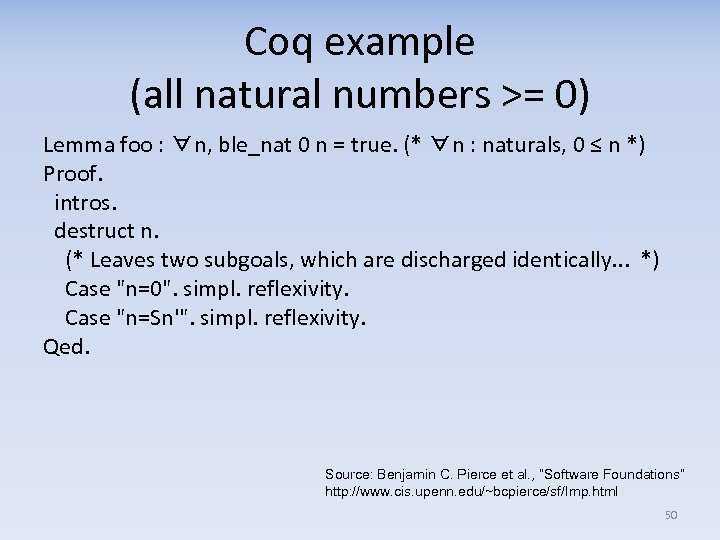Coq example (all natural numbers >= 0) Lemma foo : ∀n, ble_nat 0 n = true. (* ∀n : naturals, 0 ≤ n *) Proof. intros. destruct n. (* Leaves two subgoals, which are discharged identically. . . *) Case "n=0". simpl. reflexivity. Case "n=Sn'". simpl. reflexivity. Qed. Source: Benjamin C. Pierce et al. , “Software Foundations” http: //www. cis. upenn. edu/~bcpierce/sf/Imp. html 50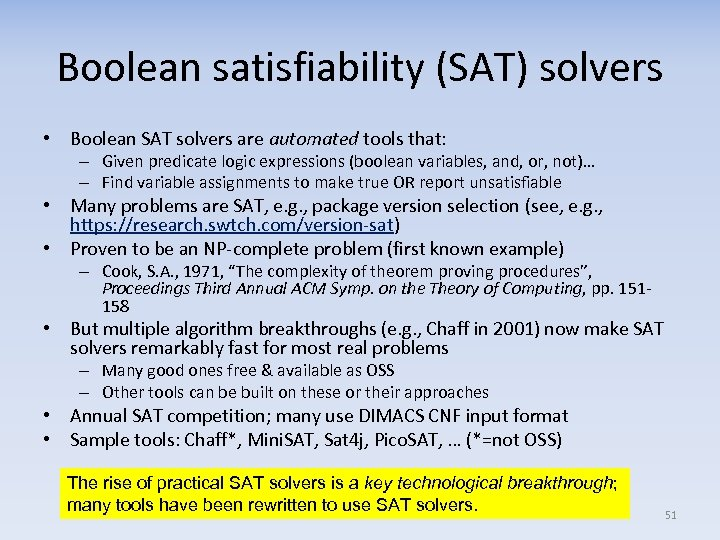Boolean satisfiability (SAT) solvers • Boolean SAT solvers are automated tools that: – Given predicate logic expressions (boolean variables, and, or, not)… – Find variable assignments to make true OR report unsatisfiable • Many problems are SAT, e. g. , package version selection (see, e. g. , https: //research. swtch. com/version-sat) • Proven to be an NP-complete problem (first known example) – Cook, S. A. , 1971, “The complexity of theorem proving procedures”, Proceedings Third Annual ACM Symp. on the Theory of Computing, pp. 151158 • But multiple algorithm breakthroughs (e. g. , Chaff in 2001) now make SAT solvers remarkably fast for most real problems – Many good ones free & available as OSS – Other tools can be built on these or their approaches • Annual SAT competition; many use DIMACS CNF input format • Sample tools: Chaff*, Mini. SAT, Sat 4 j, Pico. SAT, … (*=not OSS) The rise of practical SAT solvers is a key technological breakthrough; many tools have been rewritten to use SAT solvers. 51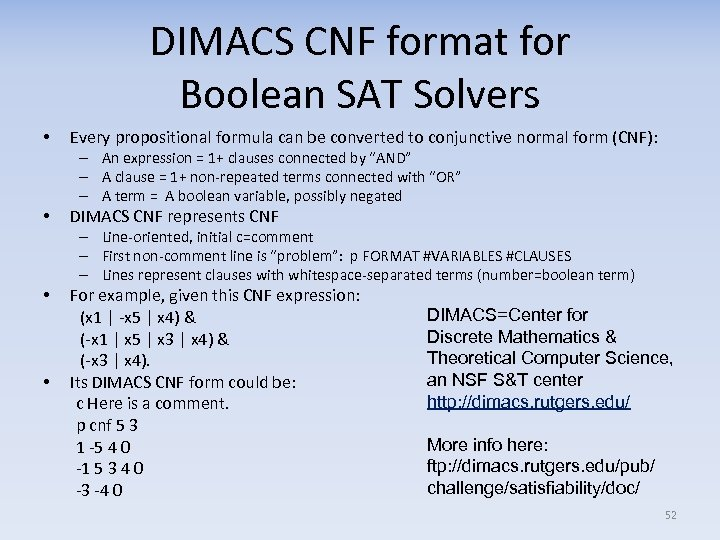DIMACS CNF format for Boolean SAT Solvers • Every propositional formula can be converted to conjunctive normal form (CNF): – An expression = 1+ clauses connected by “AND” – A clause = 1+ non-repeated terms connected with “OR” – A term = A boolean variable, possibly negated • DIMACS CNF represents CNF – Line-oriented, initial c=comment – First non-comment line is “problem”: p FORMAT #VARIABLES #CLAUSES – Lines represent clauses with whitespace-separated terms (number=boolean term) • For example, given this CNF expression: (x 1 | -x 5 | x 4) & (-x 1 | x 5 | x 3 | x 4) & (-x 3 | x 4). • Its DIMACS CNF form could be: c Here is a comment. p cnf 5 3 1 -5 4 0 -1 5 3 4 0 -3 -4 0 DIMACS=Center for Discrete Mathematics & Theoretical Computer Science, an NSF S&T center http: //dimacs. rutgers. edu/ More info here: ftp: //dimacs. rutgers. edu/pub/ challenge/satisfiability/doc/ 52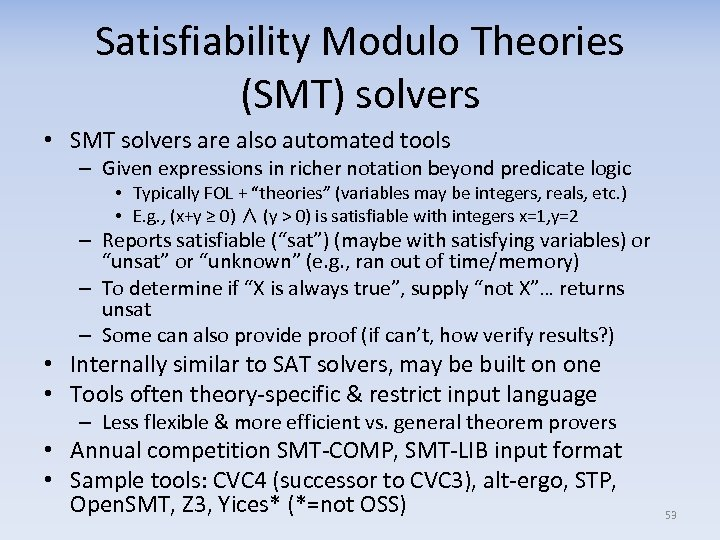Satisfiability Modulo Theories (SMT) solvers • SMT solvers are also automated tools – Given expressions in richer notation beyond predicate logic • Typically FOL + “theories” (variables may be integers, reals, etc. ) • E. g. , (x+y ≥ 0) ∧ (y > 0) is satisfiable with integers x=1, y=2 – Reports satisfiable (“sat”) (maybe with satisfying variables) or “unsat” or “unknown” (e. g. , ran out of time/memory) – To determine if “X is always true”, supply “not X”… returns unsat – Some can also provide proof (if can’t, how verify results? ) • Internally similar to SAT solvers, may be built on one • Tools often theory-specific & restrict input language – Less flexible & more efficient vs. general theorem provers • Annual competition SMT-COMP, SMT-LIB input format • Sample tools: CVC 4 (successor to CVC 3), alt-ergo, STP, Open. SMT, Z 3, Yices* (*=not OSS) 53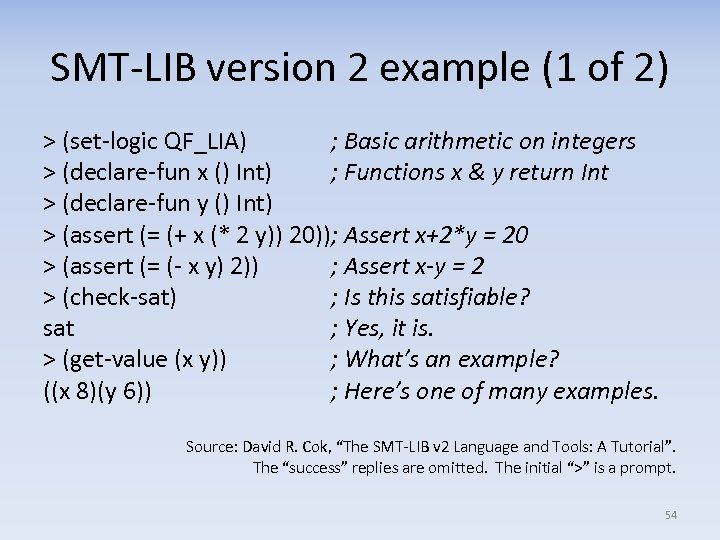SMT-LIB version 2 example (1 of 2) > (set-logic QF_LIA) ; Basic arithmetic on integers > (declare-fun x () Int) ; Functions x & y return Int > (declare-fun y () Int) > (assert (= (+ x (* 2 y)) 20)); Assert x+2*y = 20 > (assert (= (- x y) 2)) ; Assert x-y = 2 > (check-sat) ; Is this satisfiable? sat ; Yes, it is. > (get-value (x y)) ; What’s an example? ((x 8)(y 6)) ; Here’s one of many examples. Source: David R. Cok, “The SMT-LIB v 2 Language and Tools: A Tutorial”. The “success” replies are omitted. The initial “>” is a prompt. 54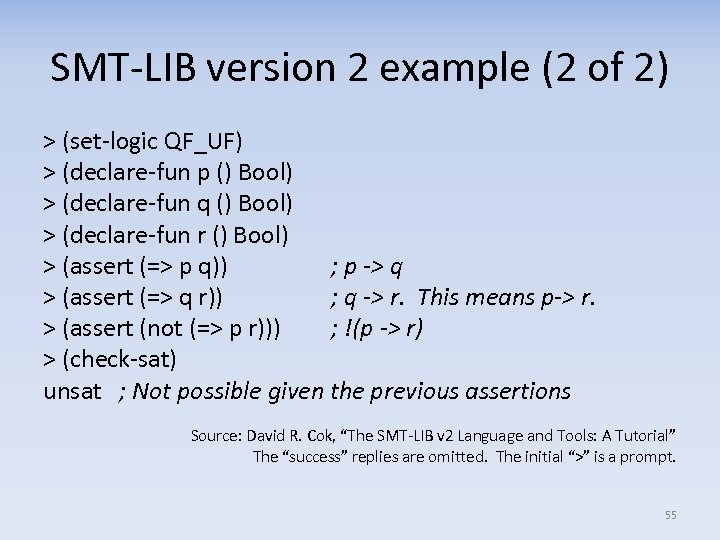SMT-LIB version 2 example (2 of 2) > (set-logic QF_UF) > (declare-fun p () Bool) > (declare-fun q () Bool) > (declare-fun r () Bool) > (assert (=> p q)) ; p -> q > (assert (=> q r)) ; q -> r. This means p-> r. > (assert (not (=> p r))) ; !(p -> r) > (check-sat) unsat ; Not possible given the previous assertions Source: David R. Cok, “The SMT-LIB v 2 Language and Tools: A Tutorial” The “success” replies are omitted. The initial “>” is a prompt. 55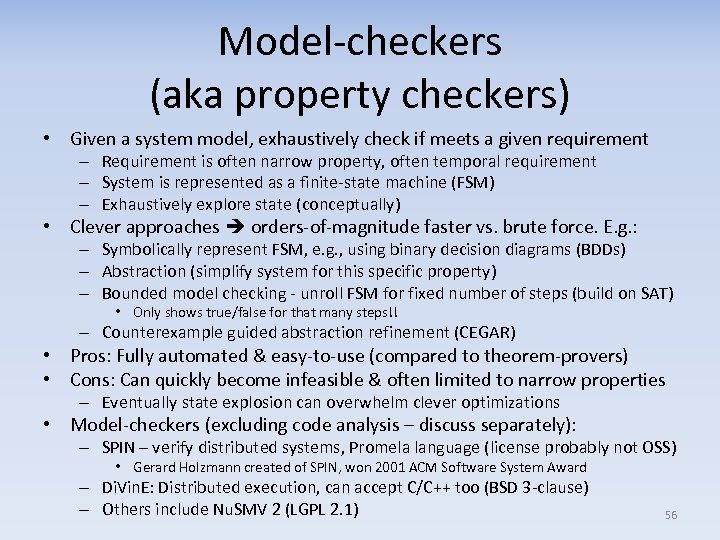Model-checkers (aka property checkers) • Given a system model, exhaustively check if meets a given requirement – Requirement is often narrow property, often temporal requirement – System is represented as a finite-state machine (FSM) – Exhaustively explore state (conceptually) • Clever approaches orders-of-magnitude faster vs. brute force. E. g. : – Symbolically represent FSM, e. g. , using binary decision diagrams (BDDs) – Abstraction (simplify system for this specific property) – Bounded model checking - unroll FSM for fixed number of steps (build on SAT) • Only shows true/false for that many steps!! – Counterexample guided abstraction refinement (CEGAR) • Pros: Fully automated & easy-to-use (compared to theorem-provers) • Cons: Can quickly become infeasible & often limited to narrow properties – Eventually state explosion can overwhelm clever optimizations • Model-checkers (excluding code analysis – discuss separately): – SPIN – verify distributed systems, Promela language (license probably not OSS) • Gerard Holzmann created of SPIN, won 2001 ACM Software System Award – Di. Vin. E: Distributed execution, can accept C/C++ too (BSD 3 -clause) – Others include Nu. SMV 2 (LGPL 2. 1) 56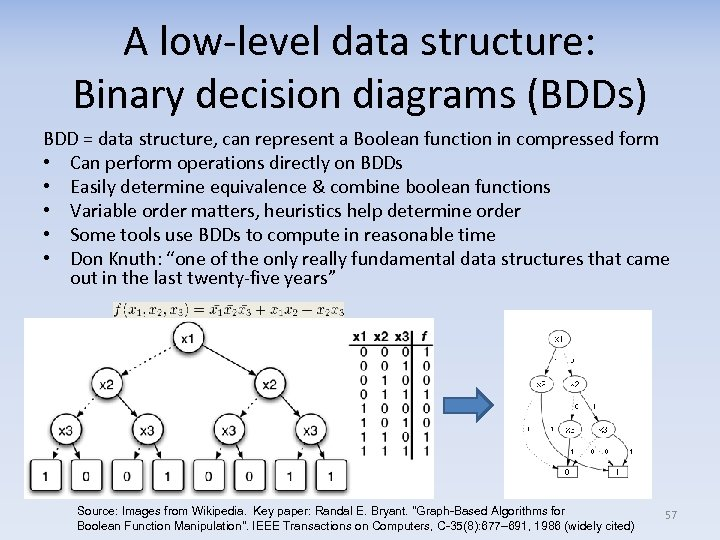A low-level data structure: Binary decision diagrams (BDDs) BDD = data structure, can represent a Boolean function in compressed form • Can perform operations directly on BDDs • Easily determine equivalence & combine boolean functions • Variable order matters, heuristics help determine order • Some tools use BDDs to compute in reasonable time • Don Knuth: “one of the only really fundamental data structures that came out in the last twenty-five years” Source: Images from Wikipedia. Key paper: Randal E. Bryant. "Graph-Based Algorithms for Boolean Function Manipulation". IEEE Transactions on Computers, C-35(8): 677– 691, 1986 (widely cited) 57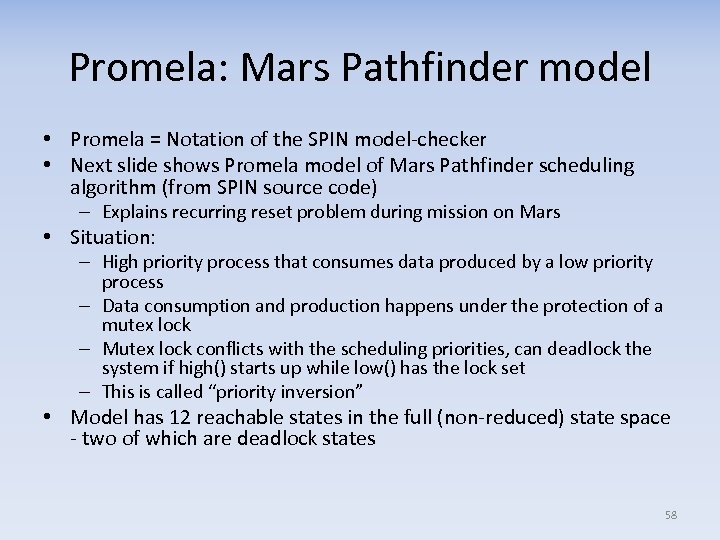Promela: Mars Pathfinder model • Promela = Notation of the SPIN model-checker • Next slide shows Promela model of Mars Pathfinder scheduling algorithm (from SPIN source code) – Explains recurring reset problem during mission on Mars • Situation: – High priority process that consumes data produced by a low priority process – Data consumption and production happens under the protection of a mutex lock – Mutex lock conflicts with the scheduling priorities, can deadlock the system if high() starts up while low() has the lock set – This is called “priority inversion” • Model has 12 reachable states in the full (non-reduced) state space - two of which are deadlock states 58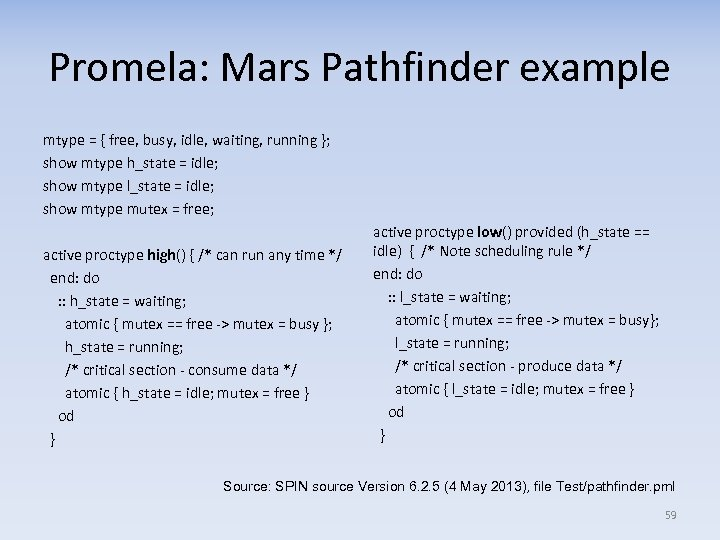Promela: Mars Pathfinder example mtype = { free, busy, idle, waiting, running }; show mtype h_state = idle; show mtype l_state = idle; show mtype mutex = free; active proctype high() { /* can run any time */ end: do : : h_state = waiting; atomic { mutex == free -> mutex = busy }; h_state = running; /* critical section - consume data */ atomic { h_state = idle; mutex = free } od } active proctype low() provided (h_state == idle) { /* Note scheduling rule */ end: do : : l_state = waiting; atomic { mutex == free -> mutex = busy}; l_state = running; /* critical section - produce data */ atomic { l_state = idle; mutex = free } od } Source: SPIN source Version 6. 2. 5 (4 May 2013), file Test/pathfinder. pml 59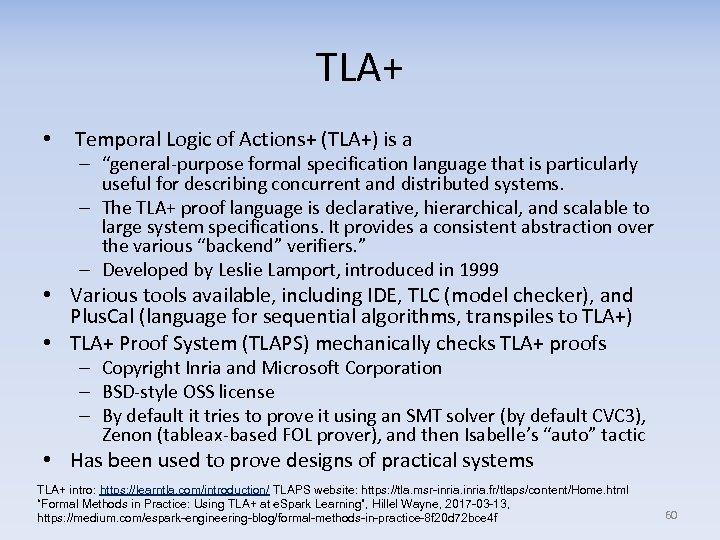TLA+ • Temporal Logic of Actions+ (TLA+) is a – “general-purpose formal specification language that is particularly useful for describing concurrent and distributed systems. – The TLA+ proof language is declarative, hierarchical, and scalable to large system specifications. It provides a consistent abstraction over the various “backend” verifiers. ” – Developed by Leslie Lamport, introduced in 1999 • Various tools available, including IDE, TLC (model checker), and Plus. Cal (language for sequential algorithms, transpiles to TLA+) • TLA+ Proof System (TLAPS) mechanically checks TLA+ proofs – Copyright Inria and Microsoft Corporation – BSD-style OSS license – By default it tries to prove it using an SMT solver (by default CVC 3), Zenon (tableax-based FOL prover), and then Isabelle’s “auto” tactic • Has been used to prove designs of practical systems TLA+ intro: https: //learntla. com/introduction/ TLAPS website: https: //tla. msr-inria. fr/tlaps/content/Home. html “Formal Methods in Practice: Using TLA+ at e. Spark Learning”, Hillel Wayne, 2017 -03 -13, https: //medium. com/espark-engineering-blog/formal-methods-in-practice-8 f 20 d 72 bce 4 f 60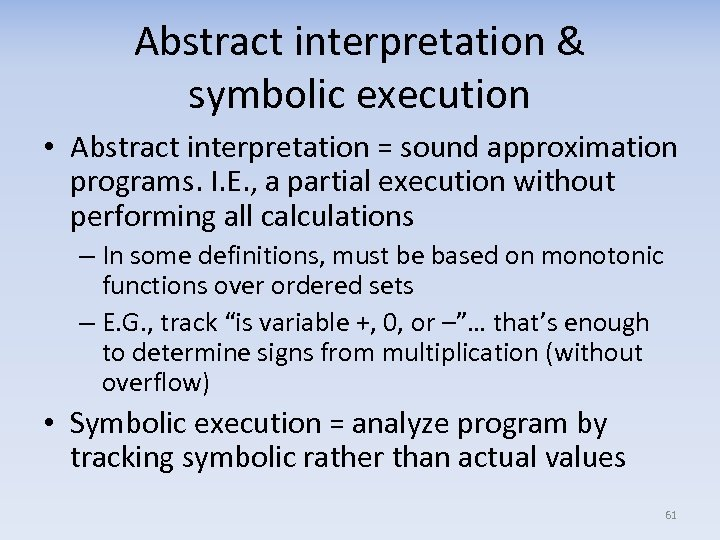Abstract interpretation & symbolic execution • Abstract interpretation = sound approximation programs. I. E. , a partial execution without performing all calculations – In some definitions, must be based on monotonic functions over ordered sets – E. G. , track “is variable +, 0, or –”… that’s enough to determine signs from multiplication (without overflow) • Symbolic execution = analyze program by tracking symbolic rather than actual values 61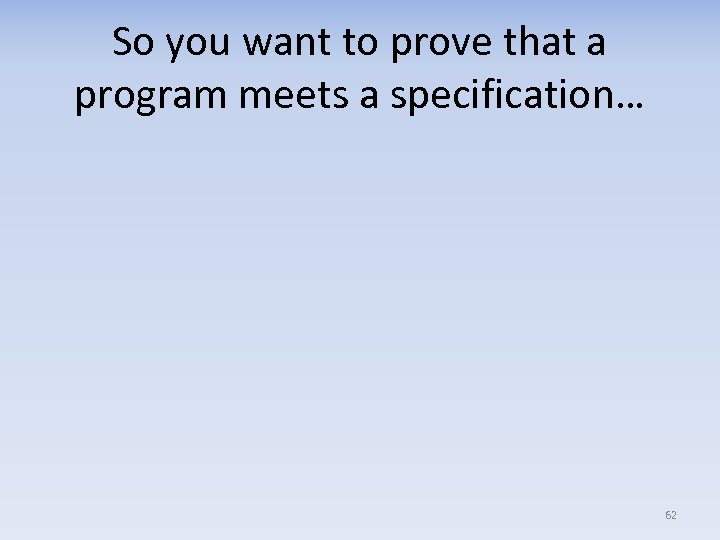So you want to prove that a program meets a specification… 62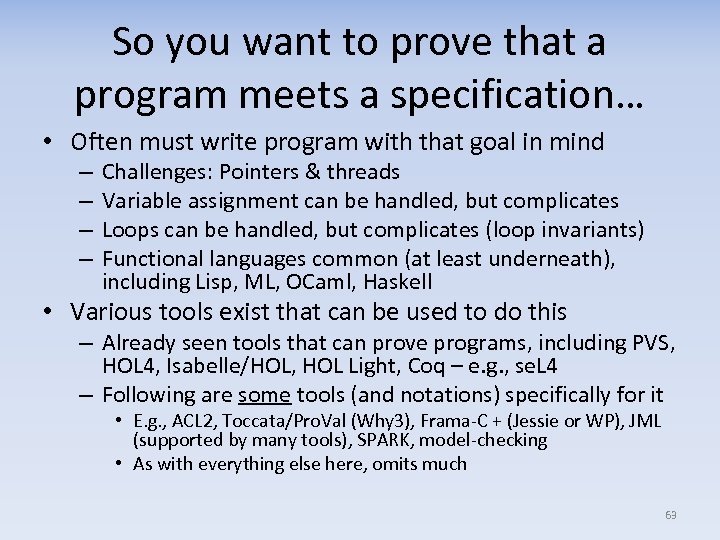So you want to prove that a program meets a specification… • Often must write program with that goal in mind – – Challenges: Pointers & threads Variable assignment can be handled, but complicates Loops can be handled, but complicates (loop invariants) Functional languages common (at least underneath), including Lisp, ML, OCaml, Haskell • Various tools exist that can be used to do this – Already seen tools that can prove programs, including PVS, HOL 4, Isabelle/HOL, HOL Light, Coq – e. g. , se. L 4 – Following are some tools (and notations) specifically for it • E. g. , ACL 2, Toccata/Pro. Val (Why 3), Frama-C + (Jessie or WP), JML (supported by many tools), SPARK, model-checking • As with everything else here, omits much 63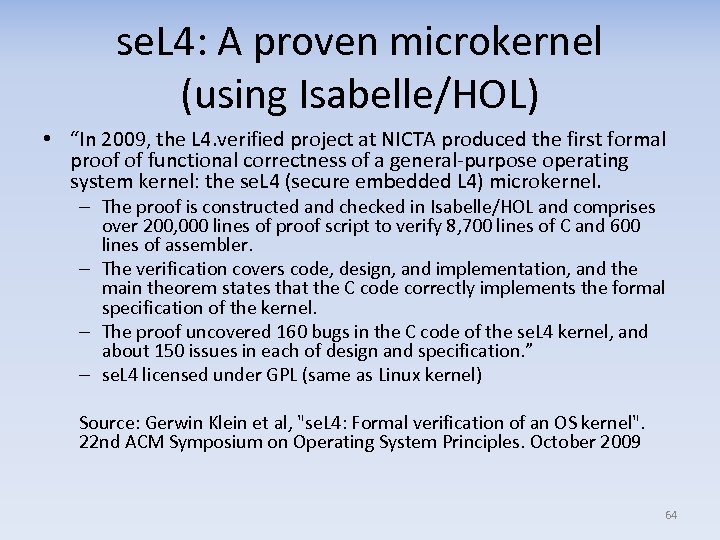se. L 4: A proven microkernel (using Isabelle/HOL) • “In 2009, the L 4. verified project at NICTA produced the first formal proof of functional correctness of a general-purpose operating system kernel: the se. L 4 (secure embedded L 4) microkernel. – The proof is constructed and checked in Isabelle/HOL and comprises over 200, 000 lines of proof script to verify 8, 700 lines of C and 600 lines of assembler. – The verification covers code, design, and implementation, and the main theorem states that the C code correctly implements the formal specification of the kernel. – The proof uncovered 160 bugs in the C code of the se. L 4 kernel, and about 150 issues in each of design and specification. ” – se. L 4 licensed under GPL (same as Linux kernel) Source: Gerwin Klein et al, "se. L 4: Formal verification of an OS kernel". 22 nd ACM Symposium on Operating System Principles. October 2009 64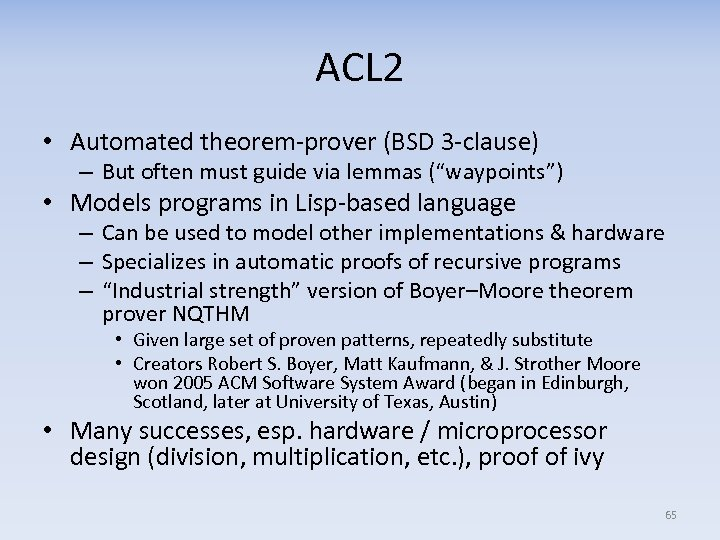ACL 2 • Automated theorem-prover (BSD 3 -clause) – But often must guide via lemmas (“waypoints”) • Models programs in Lisp-based language – Can be used to model other implementations & hardware – Specializes in automatic proofs of recursive programs – “Industrial strength” version of Boyer–Moore theorem prover NQTHM • Given large set of proven patterns, repeatedly substitute • Creators Robert S. Boyer, Matt Kaufmann, & J. Strother Moore won 2005 ACM Software System Award (began in Edinburgh, Scotland, later at University of Texas, Austin) • Many successes, esp. hardware / microprocessor design (division, multiplication, etc. ), proof of ivy 65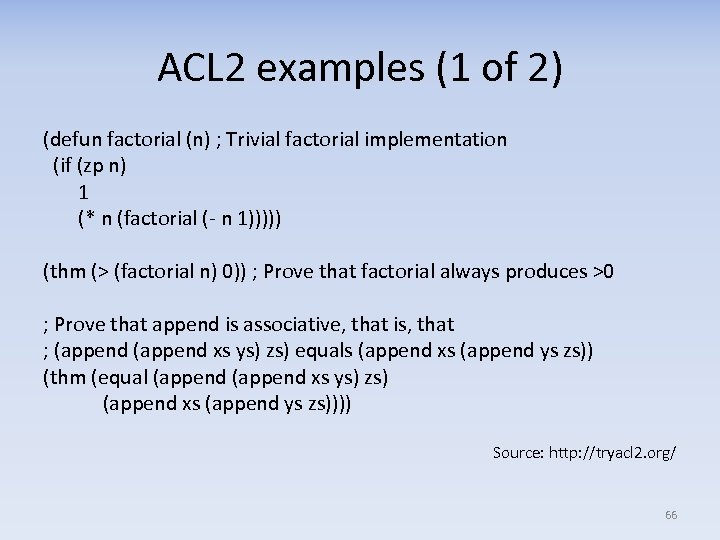ACL 2 examples (1 of 2) (defun factorial (n) ; Trivial factorial implementation (if (zp n) 1 (* n (factorial (- n 1))))) (thm (> (factorial n) 0)) ; Prove that factorial always produces >0 ; Prove that append is associative, that is, that ; (append xs ys) zs) equals (append xs (append ys zs)) (thm (equal (append xs ys) zs) (append xs (append ys zs)))) Source: http: //tryacl 2. org/ 66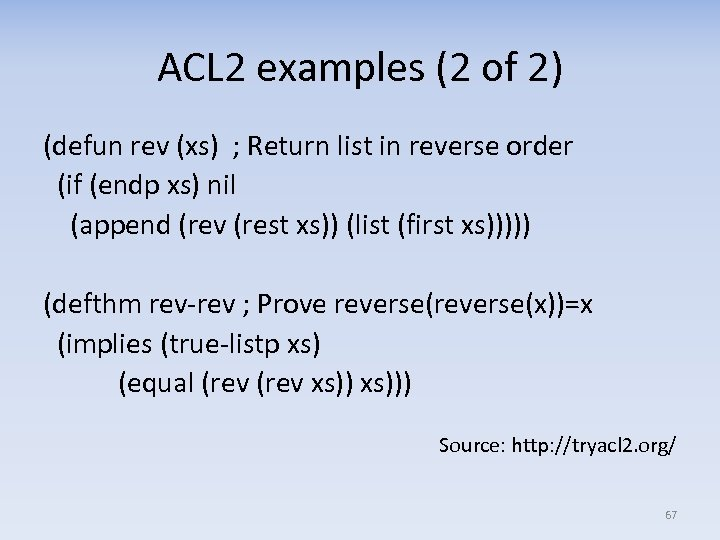ACL 2 examples (2 of 2) (defun rev (xs) ; Return list in reverse order (if (endp xs) nil (append (rev (rest xs)) (list (first xs))))) (defthm rev-rev ; Prove reverse(x))=x (implies (true-listp xs) (equal (rev xs))) Source: http: //tryacl 2. org/ 67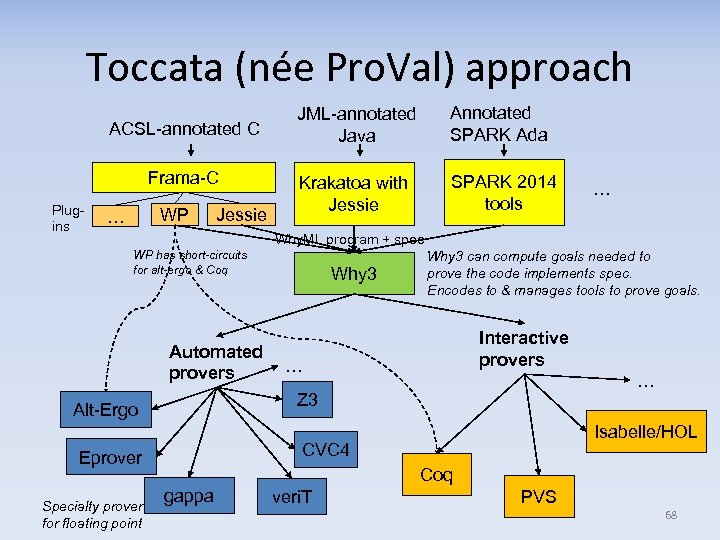Toccata (née Pro. Val) approach ACSL-annotated C Frama-C Plugins WP … Jessie JML-annotated Java Annotated SPARK Ada Krakatoa with Jessie SPARK 2014 tools … Why. ML program + spec WP has short-circuits for alt-ergo & Coq Automated provers Interactive provers … … Z 3 Alt-Ergo Isabelle/HOL CVC 4 Eprover Specialty prover for floating point Why 3 can compute goals needed to prove the code implements spec. Encodes to & manages tools to prove goals. Coq gappa veri. T PVS 68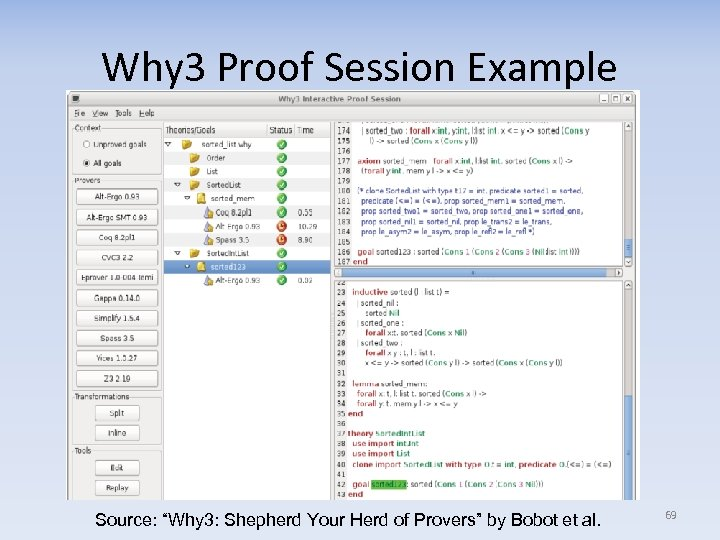Why 3 Proof Session Example Source: “Why 3: Shepherd Your Herd of Provers” by Bobot et al. 69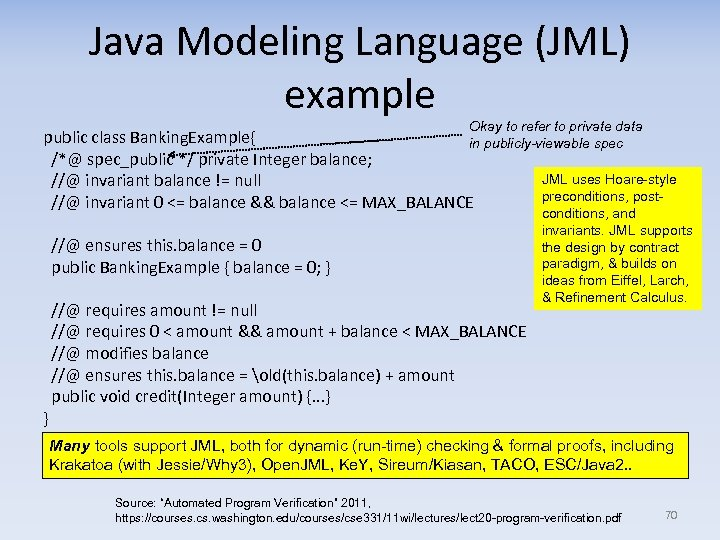Java Modeling Language (JML) example Okay to refer to private data public class Banking. Example{ in publicly-viewable spec /*@ spec_public */ private Integer balance; JML uses Hoare-style //@ invariant balance != null preconditions, post //@ invariant 0 <= balance && balance <= MAX_BALANCE //@ ensures this. balance = 0 public Banking. Example { balance = 0; } //@ requires amount != null //@ requires 0 < amount && amount + balance < MAX_BALANCE //@ modifies balance //@ ensures this. balance = old(this. balance) + amount public void credit(Integer amount) {. . . } } conditions, and invariants. JML supports the design by contract paradigm, & builds on ideas from Eiffel, Larch, & Refinement Calculus. Many tools support JML, both for dynamic (run-time) checking & formal proofs, including Krakatoa (with Jessie/Why 3), Open. JML, Ke. Y, Sireum/Kiasan, TACO, ESC/Java 2. . Source: “Automated Program Verification” 2011, https: //courses. cs. washington. edu/courses/cse 331/11 wi/lectures/lect 20 -program-verification. pdf 70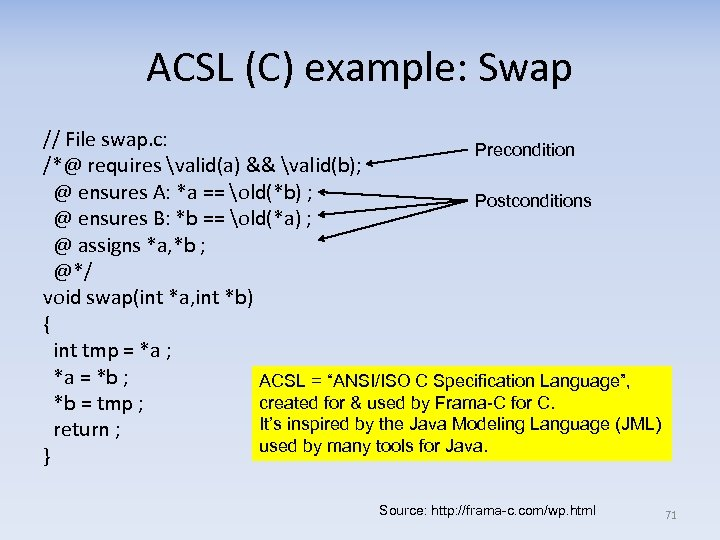ACSL (C) example: Swap // File swap. c: Precondition /*@ requires valid(a) && valid(b); @ ensures A: *a == old(*b) ; Postconditions @ ensures B: *b == old(*a) ; @ assigns *a, *b ; @*/ void swap(int *a, int *b) { int tmp = *a ; *a = *b ; ACSL = “ANSI/ISO C Specification Language”, created for & used by Frama-C for C. *b = tmp ; It’s inspired by the Java Modeling Language (JML) return ; used by many tools for Java. } Source: http: //frama-c. com/wp. html 71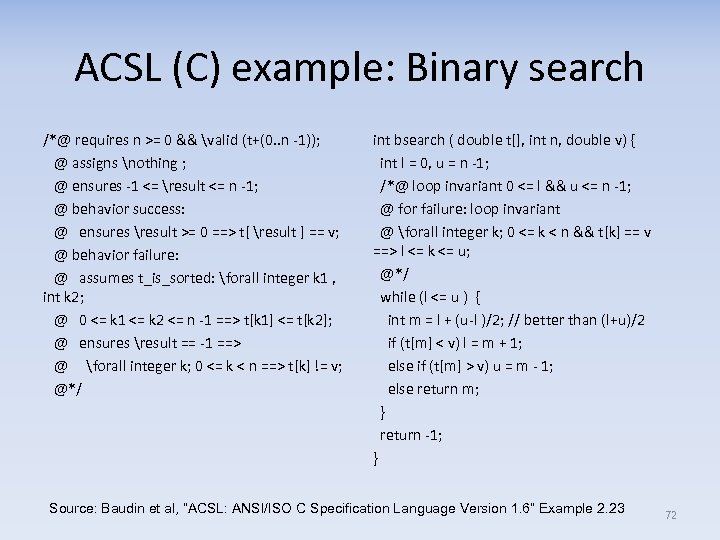ACSL (C) example: Binary search /*@ requires n >= 0 && valid (t+(0. . n -1)); @ assigns nothing ; @ ensures -1 <= result <= n -1; @ behavior success: @ ensures result >= 0 ==> t[ result ] == v; @ behavior failure: @ assumes t_is_sorted: forall integer k 1 , int k 2; @ 0 <= k 1 <= k 2 <= n -1 ==> t[k 1] <= t[k 2]; @ ensures result == -1 ==> @ forall integer k; 0 <= k < n ==> t[k] != v; @*/ int bsearch ( double t[], int n, double v) { int l = 0, u = n -1; /*@ loop invariant 0 <= l && u <= n -1; @ for failure: loop invariant @ forall integer k; 0 <= k < n && t[k] == v ==> l <= k <= u; @*/ while (l <= u ) { int m = l + (u-l )/2; // better than (l+u)/2 if (t[m] < v) l = m + 1; else if (t[m] > v) u = m - 1; else return m; } return -1; } Source: Baudin et al, “ACSL: ANSI/ISO C Specification Language Version 1. 6” Example 2. 23 72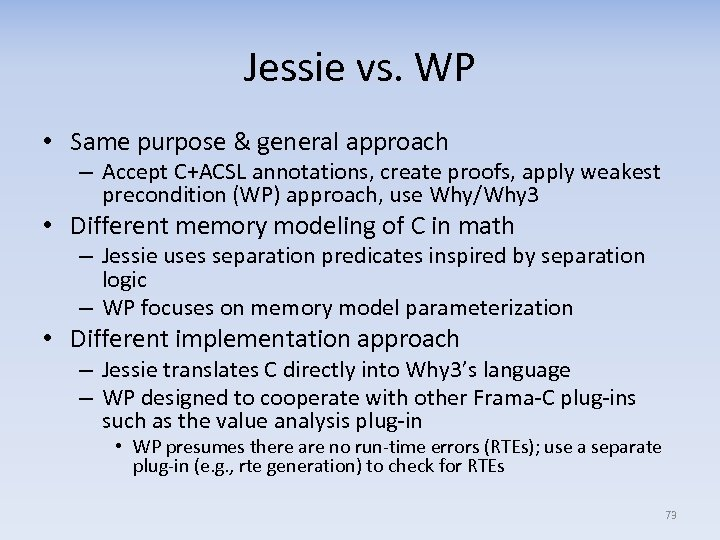Jessie vs. WP • Same purpose & general approach – Accept C+ACSL annotations, create proofs, apply weakest precondition (WP) approach, use Why/Why 3 • Different memory modeling of C in math – Jessie uses separation predicates inspired by separation logic – WP focuses on memory model parameterization • Different implementation approach – Jessie translates C directly into Why 3’s language – WP designed to cooperate with other Frama-C plug-ins such as the value analysis plug-in • WP presumes there are no run-time errors (RTEs); use a separate plug-in (e. g. , rte generation) to check for RTEs 73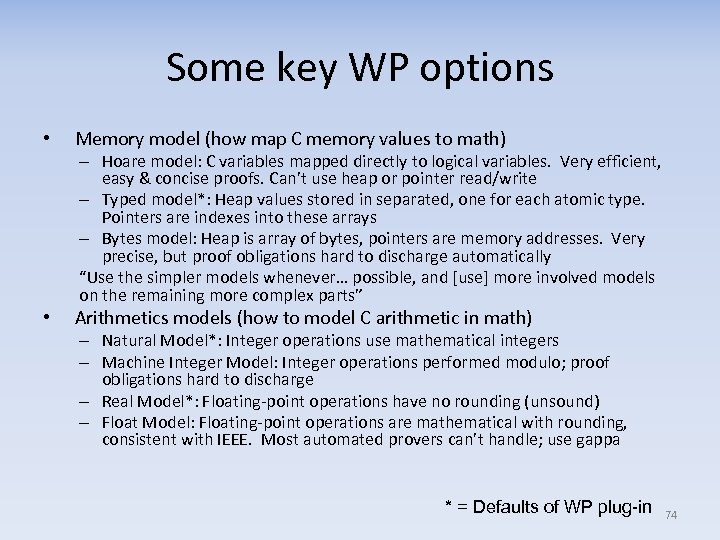Some key WP options • Memory model (how map C memory values to math) – Hoare model: C variables mapped directly to logical variables. Very efficient, easy & concise proofs. Can’t use heap or pointer read/write – Typed model*: Heap values stored in separated, one for each atomic type. Pointers are indexes into these arrays – Bytes model: Heap is array of bytes, pointers are memory addresses. Very precise, but proof obligations hard to discharge automatically “Use the simpler models whenever… possible, and [use] more involved models on the remaining more complex parts” • Arithmetics models (how to model C arithmetic in math) – Natural Model*: Integer operations use mathematical integers – Machine Integer Model: Integer operations performed modulo; proof obligations hard to discharge – Real Model*: Floating-point operations have no rounding (unsound) – Float Model: Floating-point operations are mathematical with rounding, consistent with IEEE. Most automated provers can’t handle; use gappa * = Defaults of WP plug-in 74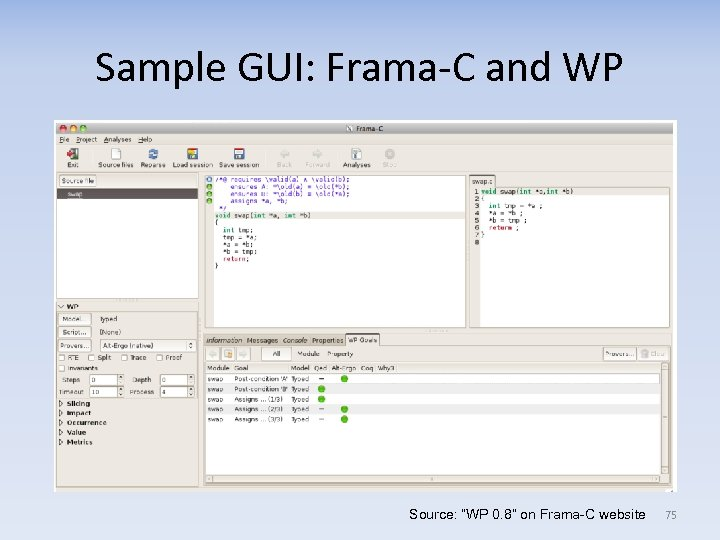Sample GUI: Frama-C and WP Source: “WP 0. 8” on Frama-C website 75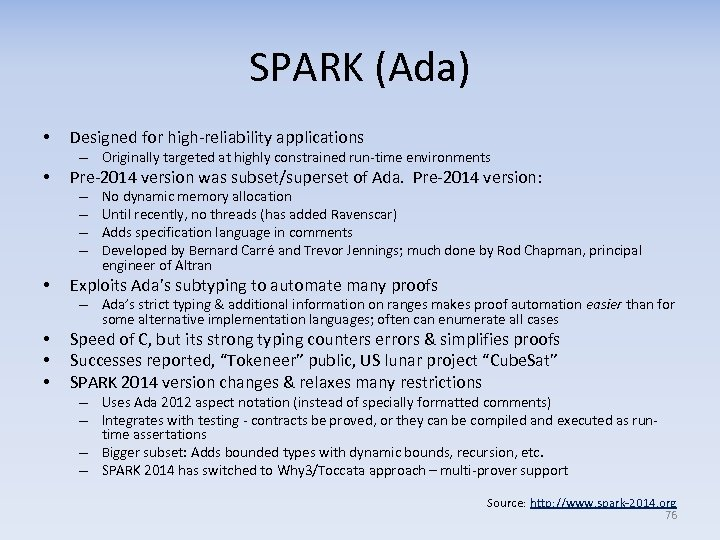SPARK (Ada) • Designed for high-reliability applications – Originally targeted at highly constrained run-time environments • Pre-2014 version was subset/superset of Ada. Pre-2014 version: – – • No dynamic memory allocation Until recently, no threads (has added Ravenscar) Adds specification language in comments Developed by Bernard Carré and Trevor Jennings; much done by Rod Chapman, principal engineer of Altran Exploits Ada’s subtyping to automate many proofs – Ada’s strict typing & additional information on ranges makes proof automation easier than for some alternative implementation languages; often can enumerate all cases • • • Speed of C, but its strong typing counters errors & simplifies proofs Successes reported, “Tokeneer” public, US lunar project “Cube. Sat” SPARK 2014 version changes & relaxes many restrictions – Uses Ada 2012 aspect notation (instead of specially formatted comments) – Integrates with testing - contracts be proved, or they can be compiled and executed as runtime assertations – Bigger subset: Adds bounded types with dynamic bounds, recursion, etc. – SPARK 2014 has switched to Why 3/Toccata approach – multi-prover support Source: http: //www. spark-2014. org 76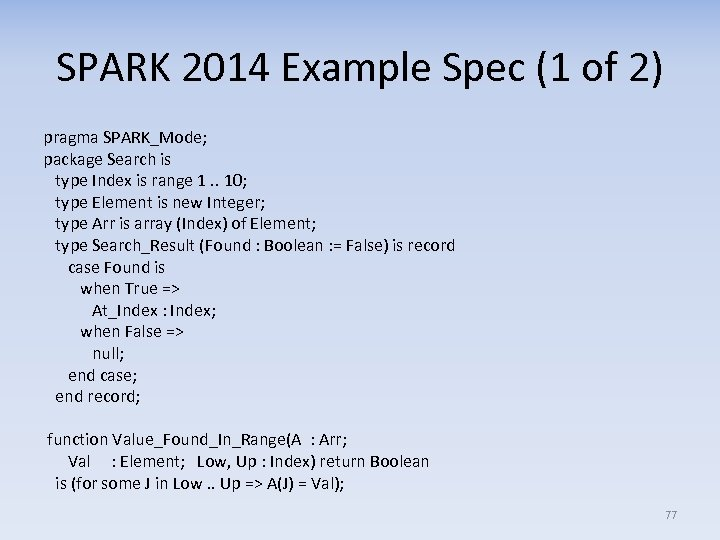SPARK 2014 Example Spec (1 of 2) pragma SPARK_Mode; package Search is type Index is range 1. . 10; type Element is new Integer; type Arr is array (Index) of Element; type Search_Result (Found : Boolean : = False) is record case Found is when True => At_Index : Index; when False => null; end case; end record; function Value_Found_In_Range(A : Arr; Val : Element; Low, Up : Index) return Boolean is (for some J in Low. . Up => A(J) = Val); 77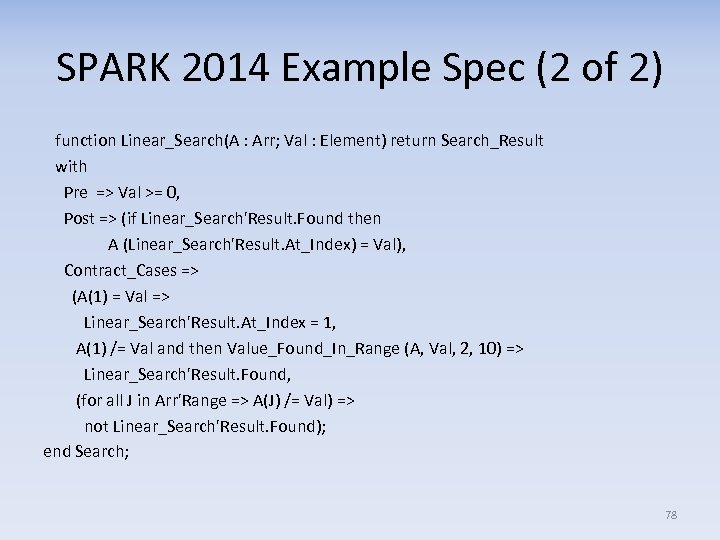SPARK 2014 Example Spec (2 of 2) function Linear_Search(A : Arr; Val : Element) return Search_Result with Pre => Val >= 0, Post => (if Linear_Search'Result. Found then A (Linear_Search'Result. At_Index) = Val), Contract_Cases => (A(1) = Val => Linear_Search'Result. At_Index = 1, A(1) /= Val and then Value_Found_In_Range (A, Val, 2, 10) => Linear_Search'Result. Found, (for all J in Arr'Range => A(J) /= Val) => not Linear_Search'Result. Found); end Search; 78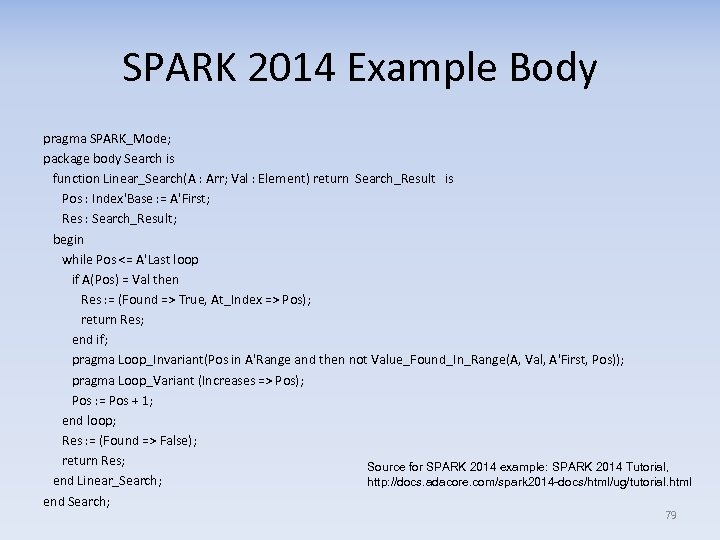SPARK 2014 Example Body pragma SPARK_Mode; package body Search is function Linear_Search(A : Arr; Val : Element) return Search_Result is Pos : Index'Base : = A'First; Res : Search_Result; begin while Pos <= A'Last loop if A(Pos) = Val then Res : = (Found => True, At_Index => Pos); return Res; end if; pragma Loop_Invariant(Pos in A'Range and then not Value_Found_In_Range(A, Val, A'First, Pos)); pragma Loop_Variant (Increases => Pos); Pos : = Pos + 1; end loop; Res : = (Found => False); return Res; Source for SPARK 2014 example: SPARK 2014 Tutorial, end Linear_Search; http: //docs. adacore. com/spark 2014 -docs/html/ug/tutorial. html end Search; 79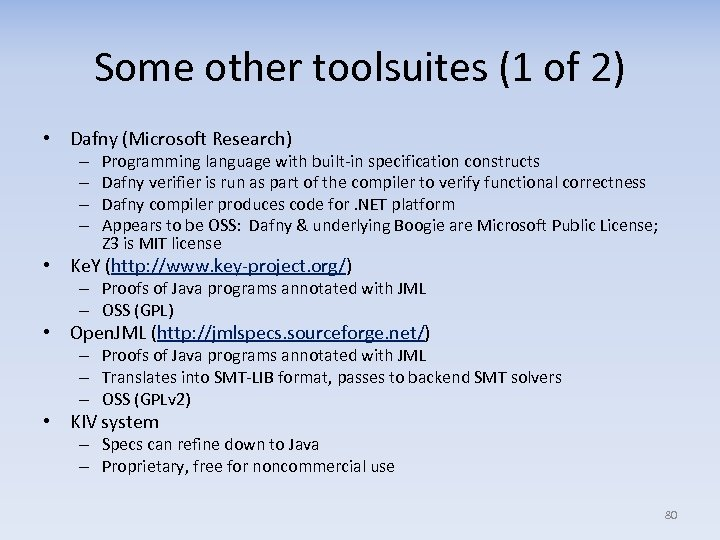Some other toolsuites (1 of 2) • Dafny (Microsoft Research) – – Programming language with built-in specification constructs Dafny verifier is run as part of the compiler to verify functional correctness Dafny compiler produces code for. NET platform Appears to be OSS: Dafny & underlying Boogie are Microsoft Public License; Z 3 is MIT license • Ke. Y (http: //www. key-project. org/) – Proofs of Java programs annotated with JML – OSS (GPL) • Open. JML (http: //jmlspecs. sourceforge. net/) – Proofs of Java programs annotated with JML – Translates into SMT-LIB format, passes to backend SMT solvers – OSS (GPLv 2) • KIV system – Specs can refine down to Java – Proprietary, free for noncommercial use 80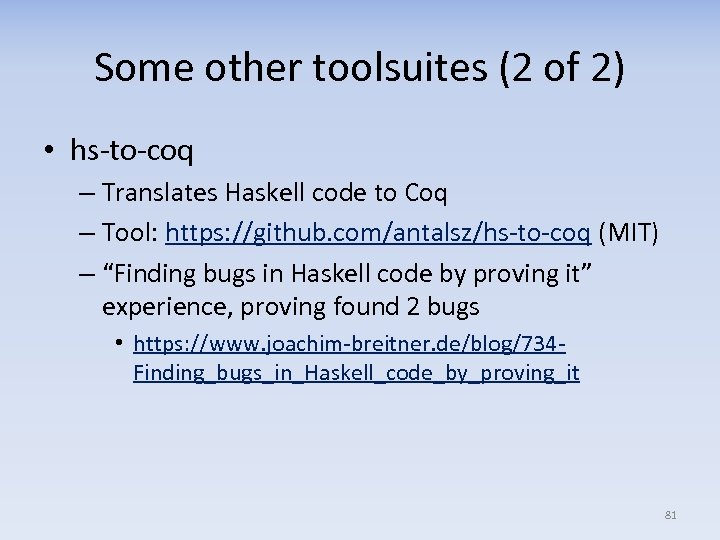Some other toolsuites (2 of 2) • hs-to-coq – Translates Haskell code to Coq – Tool: https: //github. com/antalsz/hs-to-coq (MIT) – “Finding bugs in Haskell code by proving it” experience, proving found 2 bugs • https: //www. joachim-breitner. de/blog/734 Finding_bugs_in_Haskell_code_by_proving_it 81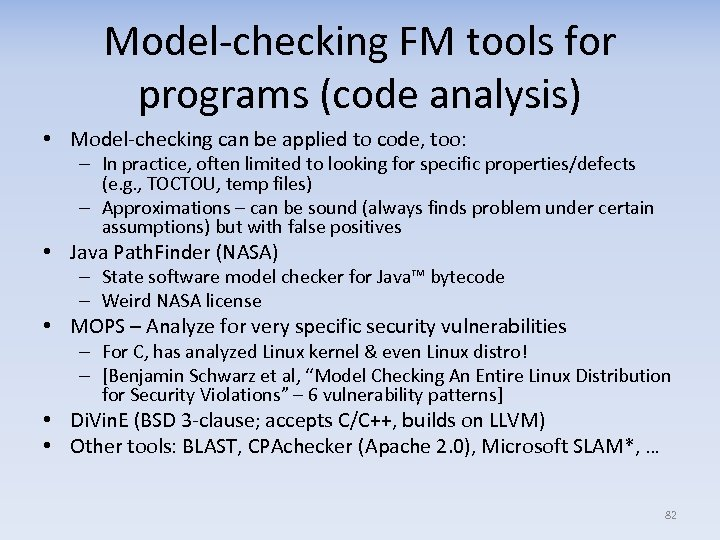Model-checking FM tools for programs (code analysis) • Model-checking can be applied to code, too: – In practice, often limited to looking for specific properties/defects (e. g. , TOCTOU, temp files) – Approximations – can be sound (always finds problem under certain assumptions) but with false positives • Java Path. Finder (NASA) – State software model checker for Java™ bytecode – Weird NASA license • MOPS – Analyze for very specific security vulnerabilities – For C, has analyzed Linux kernel & even Linux distro! – [Benjamin Schwarz et al, “Model Checking An Entire Linux Distribution for Security Violations” – 6 vulnerability patterns] • Di. Vin. E (BSD 3 -clause; accepts C/C++, builds on LLVM) • Other tools: BLAST, CPAchecker (Apache 2. 0), Microsoft SLAM*, … 82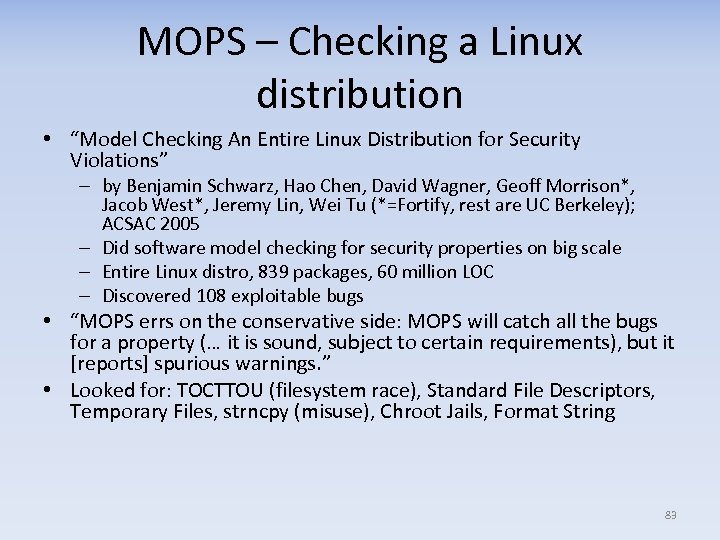MOPS – Checking a Linux distribution • “Model Checking An Entire Linux Distribution for Security Violations” – by Benjamin Schwarz, Hao Chen, David Wagner, Geoff Morrison*, Jacob West*, Jeremy Lin, Wei Tu (*=Fortify, rest are UC Berkeley); ACSAC 2005 – Did software model checking for security properties on big scale – Entire Linux distro, 839 packages, 60 million LOC – Discovered 108 exploitable bugs • “MOPS errs on the conservative side: MOPS will catch all the bugs for a property (… it is sound, subject to certain requirements), but it [reports] spurious warnings. ” • Looked for: TOCTTOU (filesystem race), Standard File Descriptors, Temporary Files, strncpy (misuse), Chroot Jails, Format String 83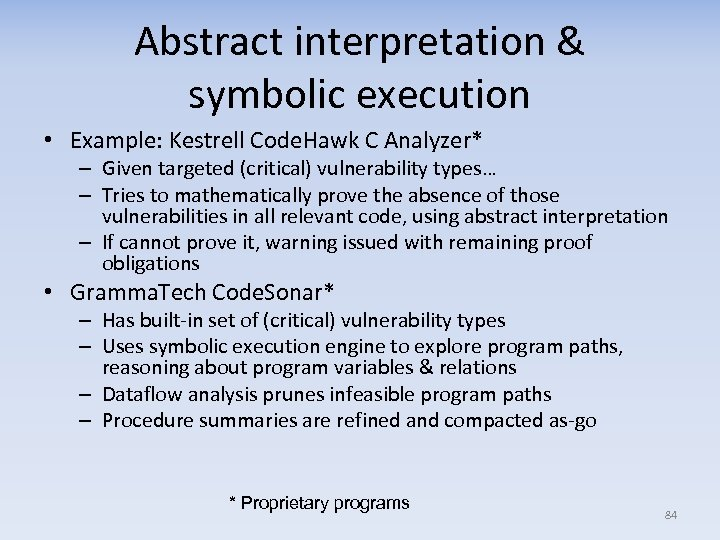Abstract interpretation & symbolic execution • Example: Kestrell Code. Hawk C Analyzer* – Given targeted (critical) vulnerability types… – Tries to mathematically prove the absence of those vulnerabilities in all relevant code, using abstract interpretation – If cannot prove it, warning issued with remaining proof obligations • Gramma. Tech Code. Sonar* – Has built-in set of (critical) vulnerability types – Uses symbolic execution engine to explore program paths, reasoning about program variables & relations – Dataflow analysis prunes infeasible program paths – Procedure summaries are refined and compacted as-go * Proprietary programs 84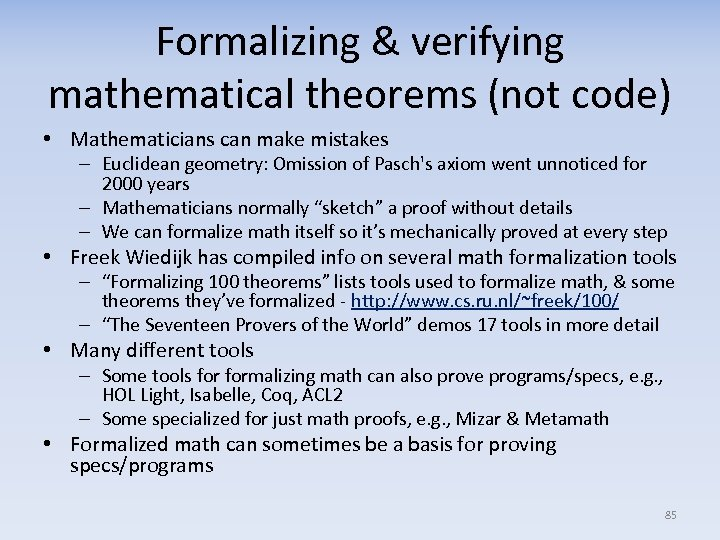Formalizing & verifying mathematical theorems (not code) • Mathematicians can make mistakes – Euclidean geometry: Omission of Pasch's axiom went unnoticed for 2000 years – Mathematicians normally “sketch” a proof without details – We can formalize math itself so it’s mechanically proved at every step • Freek Wiedijk has compiled info on several math formalization tools – “Formalizing 100 theorems” lists tools used to formalize math, & some theorems they’ve formalized - http: //www. cs. ru. nl/~freek/100/ – “The Seventeen Provers of the World” demos 17 tools in more detail • Many different tools – Some tools formalizing math can also prove programs/specs, e. g. , HOL Light, Isabelle, Coq, ACL 2 – Some specialized for just math proofs, e. g. , Mizar & Metamath • Formalized math can sometimes be a basis for proving specs/programs 85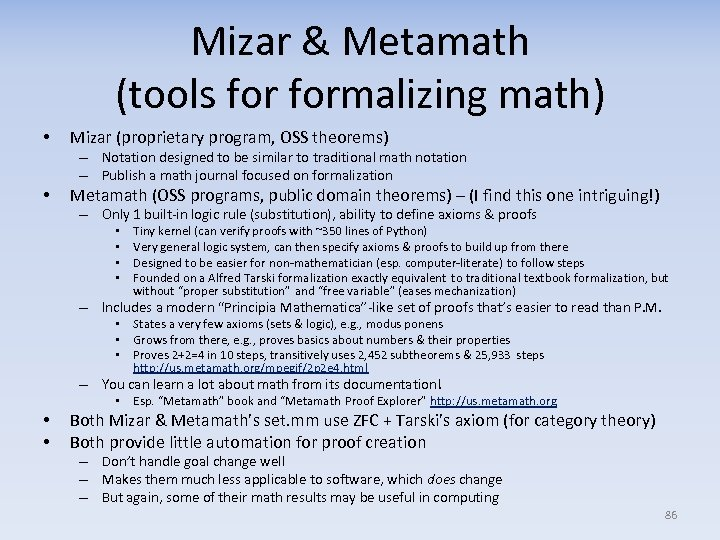Mizar & Metamath (tools formalizing math) • Mizar (proprietary program, OSS theorems) – Notation designed to be similar to traditional math notation – Publish a math journal focused on formalization • Metamath (OSS programs, public domain theorems) – (I find this one intriguing!) – Only 1 built-in logic rule (substitution), ability to define axioms & proofs • • Tiny kernel (can verify proofs with ~350 lines of Python) Very general logic system, can then specify axioms & proofs to build up from there Designed to be easier for non-mathematician (esp. computer-literate) to follow steps Founded on a Alfred Tarski formalization exactly equivalent to traditional textbook formalization, but without “proper substitution” and “free variable” (eases mechanization) – Includes a modern “Principia Mathematica”-like set of proofs that’s easier to read than P. M. • States a very few axioms (sets & logic), e. g. , modus ponens • Grows from there, e. g. , proves basics about numbers & their properties • Proves 2+2=4 in 10 steps, transitively uses 2, 452 subtheorems & 25, 933 steps http: //us. metamath. org/mpegif/2 p 2 e 4. html – You can learn a lot about math from its documentation! • Esp. “Metamath” book and “Metamath Proof Explorer” http: //us. metamath. org • • Both Mizar & Metamath’s set. mm use ZFC + Tarski’s axiom (for category theory) Both provide little automation for proof creation – Don’t handle goal change well – Makes them much less applicable to software, which does change – But again, some of their math results may be useful in computing 86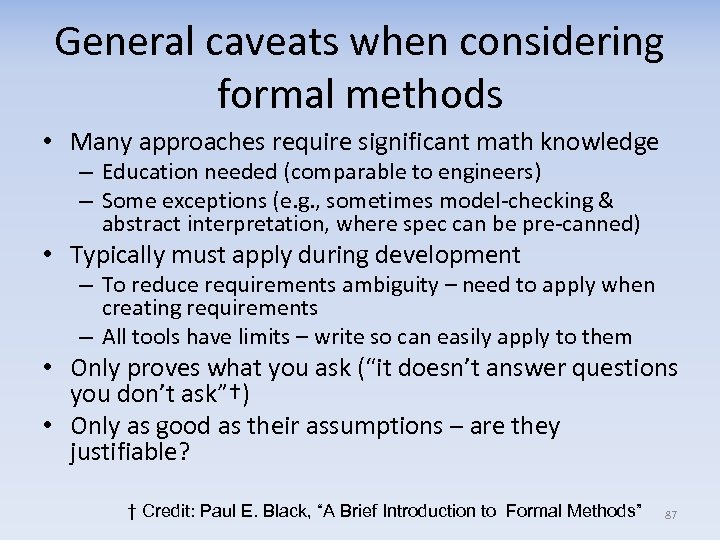General caveats when considering formal methods • Many approaches require significant math knowledge – Education needed (comparable to engineers) – Some exceptions (e. g. , sometimes model-checking & abstract interpretation, where spec can be pre-canned) • Typically must apply during development – To reduce requirements ambiguity – need to apply when creating requirements – All tools have limits – write so can easily apply to them • Only proves what you ask (“it doesn’t answer questions you don’t ask”†) • Only as good as their assumptions – are they justifiable? † Credit: Paul E. Black, “A Brief Introduction to Formal Methods” 87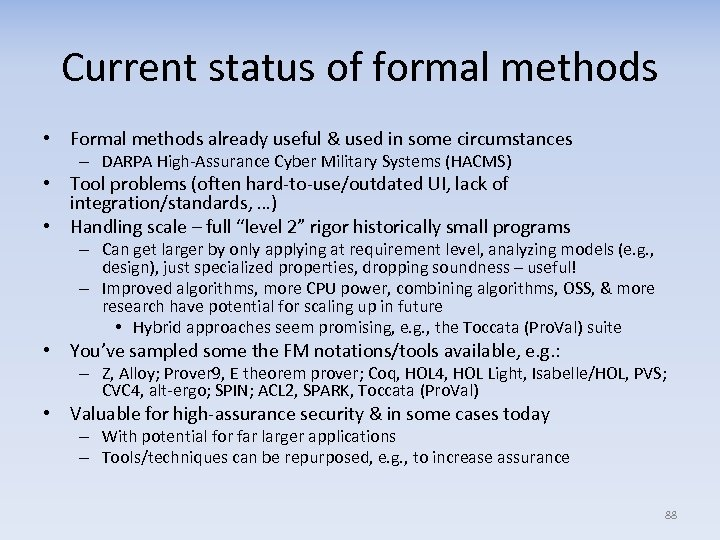Current status of formal methods • Formal methods already useful & used in some circumstances – DARPA High-Assurance Cyber Military Systems (HACMS) • Tool problems (often hard-to-use/outdated UI, lack of integration/standards, …) • Handling scale – full “level 2” rigor historically small programs – Can get larger by only applying at requirement level, analyzing models (e. g. , design), just specialized properties, dropping soundness – useful! – Improved algorithms, more CPU power, combining algorithms, OSS, & more research have potential for scaling up in future • Hybrid approaches seem promising, e. g. , the Toccata (Pro. Val) suite • You’ve sampled some the FM notations/tools available, e. g. : – Z, Alloy; Prover 9, E theorem prover; Coq, HOL 4, HOL Light, Isabelle/HOL, PVS; CVC 4, alt-ergo; SPIN; ACL 2, SPARK, Toccata (Pro. Val) • Valuable for high-assurance security & in some cases today – With potential for far larger applications – Tools/techniques can be repurposed, e. g. , to increase assurance 88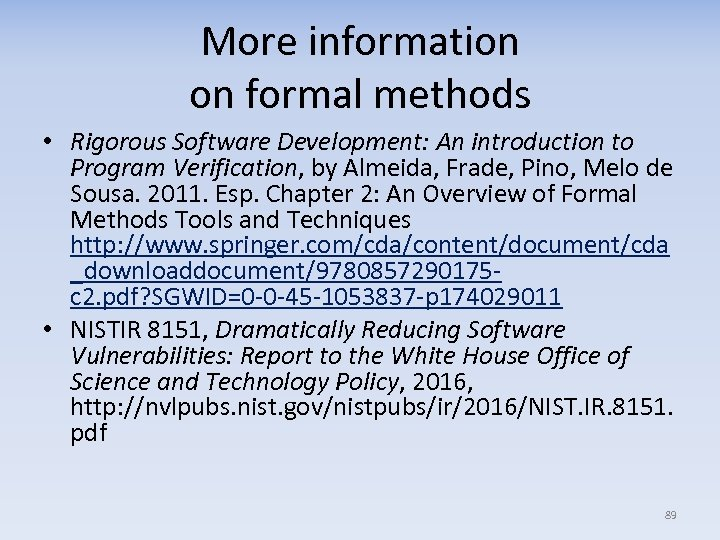More information on formal methods • Rigorous Software Development: An introduction to Program Verification, by Almeida, Frade, Pino, Melo de Sousa. 2011. Esp. Chapter 2: An Overview of Formal Methods Tools and Techniques http: //www. springer. com/cda/content/document/cda _downloaddocument/9780857290175 c 2. pdf? SGWID=0 -0 -45 -1053837 -p 174029011 • NISTIR 8151, Dramatically Reducing Software Vulnerabilities: Report to the White House Office of Science and Technology Policy, 2016, http: //nvlpubs. nist. gov/nistpubs/ir/2016/NIST. IR. 8151. pdf 89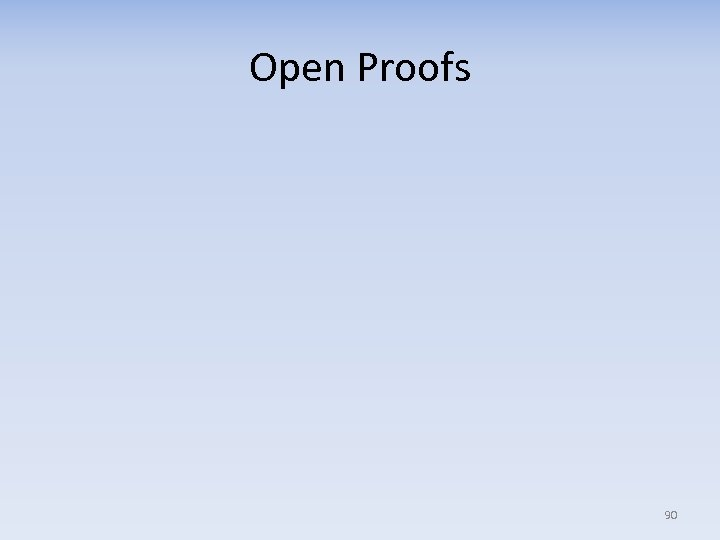Open Proofs 90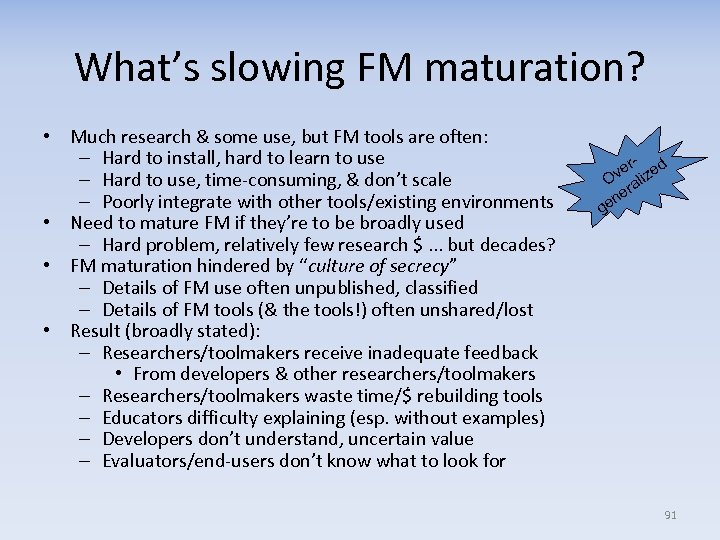What’s slowing FM maturation? • Much research & some use, but FM tools are often: – Hard to install, hard to learn to use – Hard to use, time-consuming, & don’t scale – Poorly integrate with other tools/existing environments • Need to mature FM if they’re to be broadly used – Hard problem, relatively few research \$. . . but decades? • FM maturation hindered by “culture of secrecy” – Details of FM use often unpublished, classified – Details of FM tools (& the tools!) often unshared/lost • Result (broadly stated): – Researchers/toolmakers receive inadequate feedback • From developers & other researchers/toolmakers – Researchers/toolmakers waste time/\$ rebuilding tools – Educators difficulty explaining (esp. without examples) – Developers don’t understand, uncertain value – Evaluators/end-users don’t know what to look for rve lized O ra e en g 91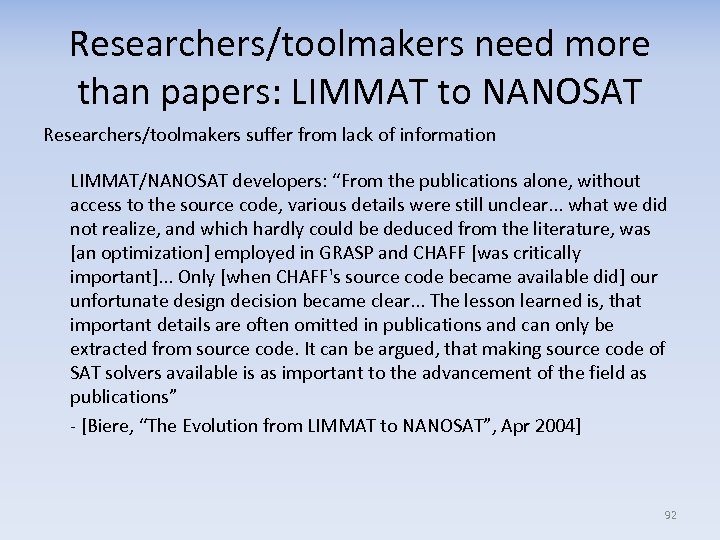Researchers/toolmakers need more than papers: LIMMAT to NANOSAT Researchers/toolmakers suffer from lack of information LIMMAT/NANOSAT developers: “From the publications alone, without access to the source code, various details were still unclear. . . what we did not realize, and which hardly could be deduced from the literature, was [an optimization] employed in GRASP and CHAFF [was critically important]. . . Only [when CHAFF's source code became available did] our unfortunate design decision became clear. . . The lesson learned is, that important details are often omitted in publications and can only be extracted from source code. It can be argued, that making source code of SAT solvers available is as important to the advancement of the field as publications” - [Biere, “The Evolution from LIMMAT to NANOSAT”, Apr 2004] 92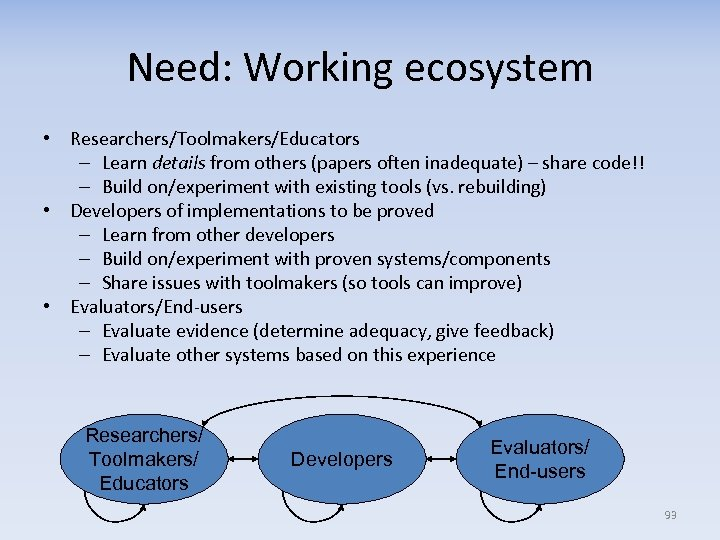Need: Working ecosystem • Researchers/Toolmakers/Educators – Learn details from others (papers often inadequate) – share code!! – Build on/experiment with existing tools (vs. rebuilding) • Developers of implementations to be proved – Learn from other developers – Build on/experiment with proven systems/components – Share issues with toolmakers (so tools can improve) • Evaluators/End-users – Evaluate evidence (determine adequacy, give feedback) – Evaluate other systems based on this experience Researchers/ Toolmakers/ Educators Developers Evaluators/ End-users 93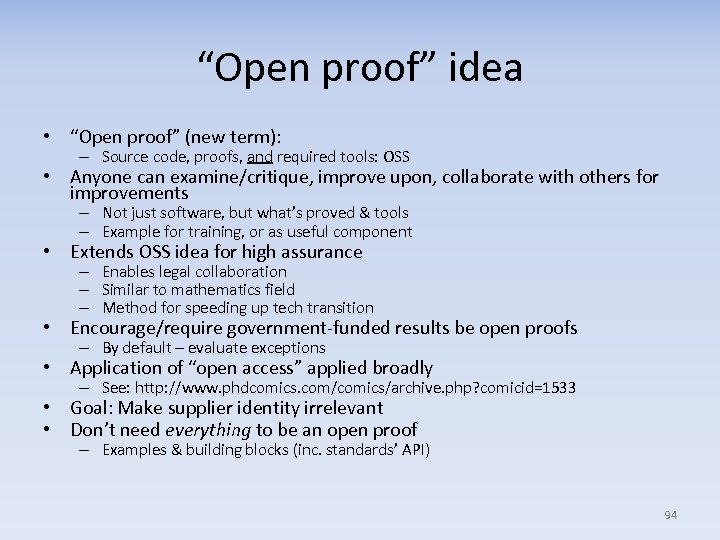“Open proof” idea • “Open proof” (new term): – Source code, proofs, and required tools: OSS • Anyone can examine/critique, improve upon, collaborate with others for improvements – Not just software, but what’s proved & tools – Example for training, or as useful component • Extends OSS idea for high assurance – Enables legal collaboration – Similar to mathematics field – Method for speeding up tech transition • Encourage/require government-funded results be open proofs – By default – evaluate exceptions • Application of “open access” applied broadly – See: http: //www. phdcomics. com/comics/archive. php? comicid=1533 • Goal: Make supplier identity irrelevant • Don’t need everything to be an open proof – Examples & building blocks (inc. standards’ API) 94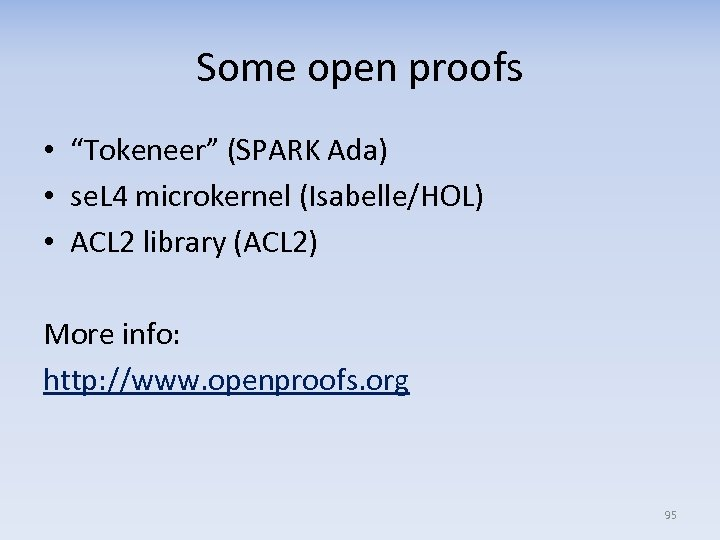Some open proofs • “Tokeneer” (SPARK Ada) • se. L 4 microkernel (Isabelle/HOL) • ACL 2 library (ACL 2) More info: http: //www. openproofs. org 95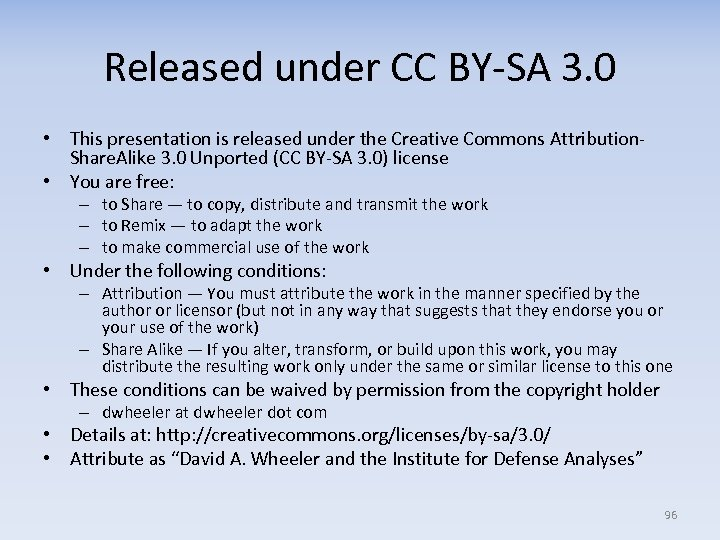Released under CC BY-SA 3. 0 • This presentation is released under the Creative Commons Attribution. Share. Alike 3. 0 Unported (CC BY-SA 3. 0) license • You are free: – to Share — to copy, distribute and transmit the work – to Remix — to adapt the work – to make commercial use of the work • Under the following conditions: – Attribution — You must attribute the work in the manner specified by the author or licensor (but not in any way that suggests that they endorse you or your use of the work) – Share Alike — If you alter, transform, or build upon this work, you may distribute the resulting work only under the same or similar license to this one • These conditions can be waived by permission from the copyright holder – dwheeler at dwheeler dot com • Details at: http: //creativecommons. org/licenses/by-sa/3. 0/ • Attribute as “David A. Wheeler and the Institute for Defense Analyses” 96